Successfully reported this slideshow.
We use your LinkedIn profile and activity data to personalize ads and to show you more relevant ads. You can change your ad preferences anytime.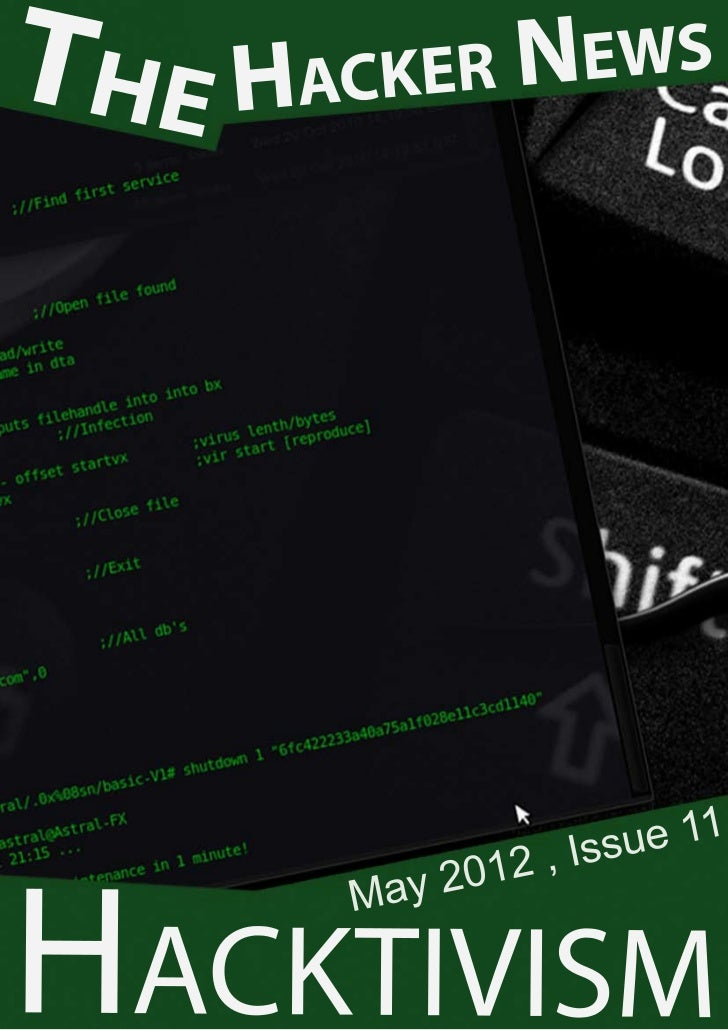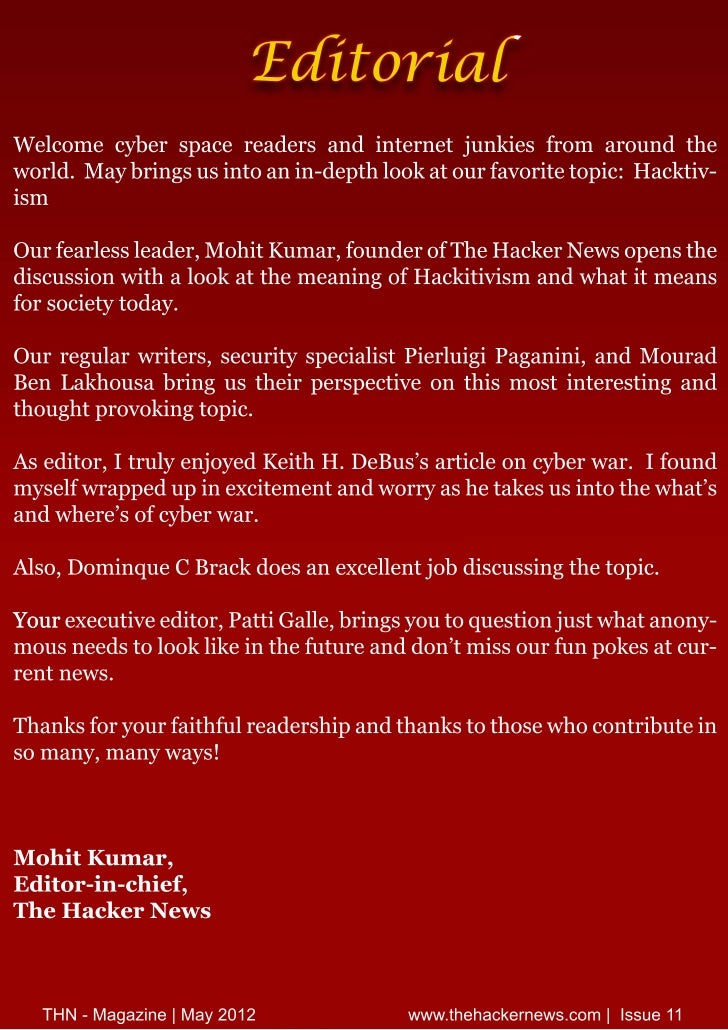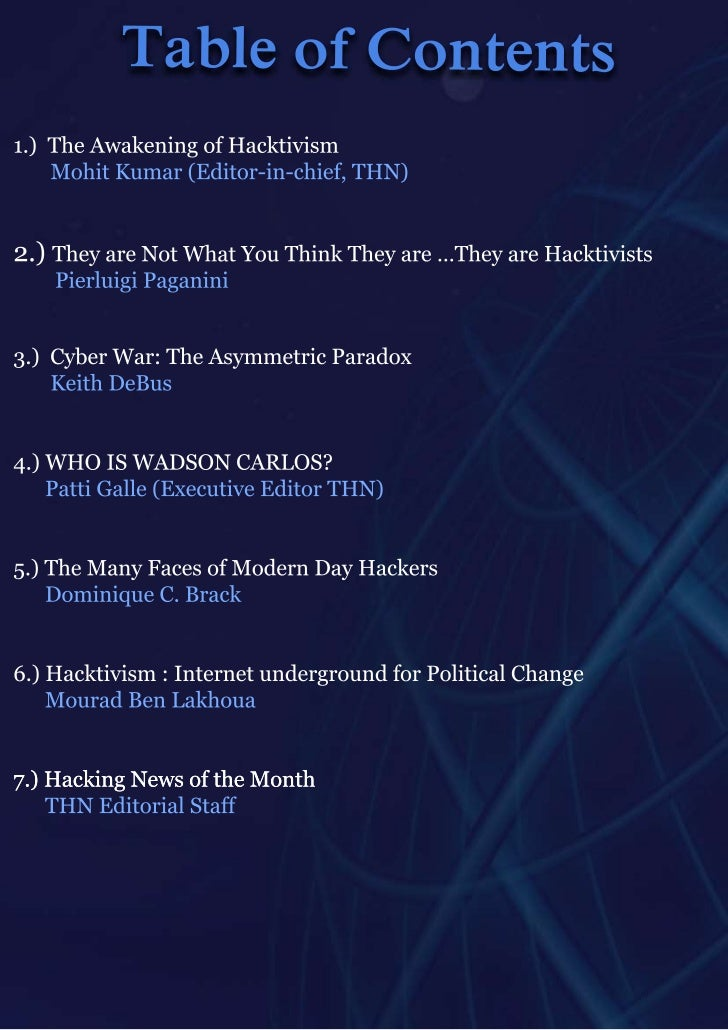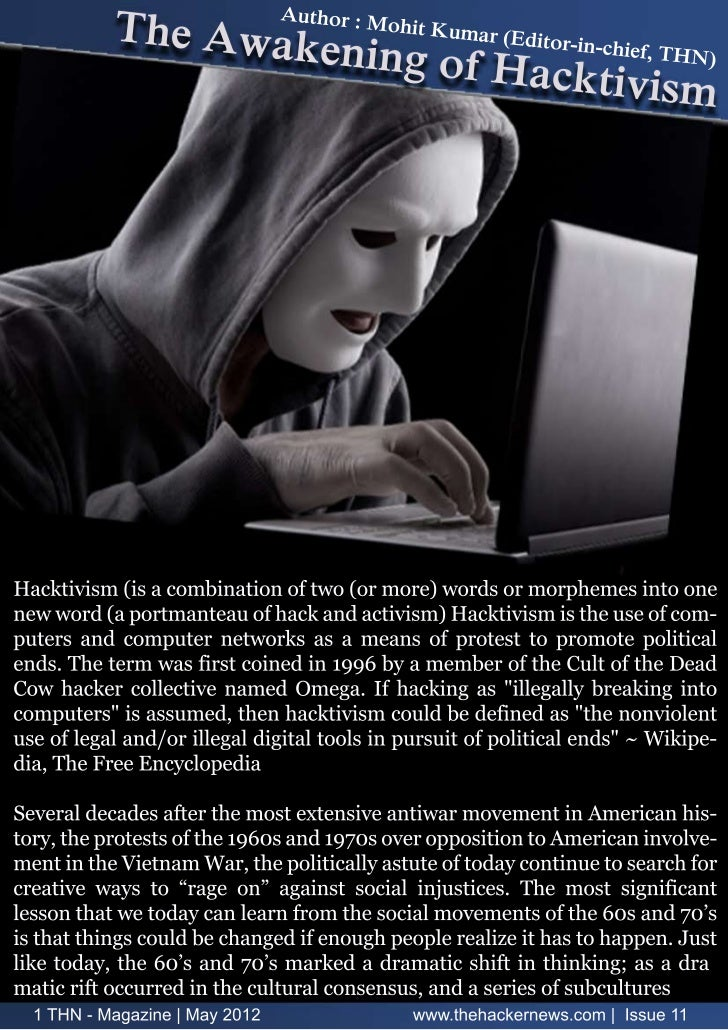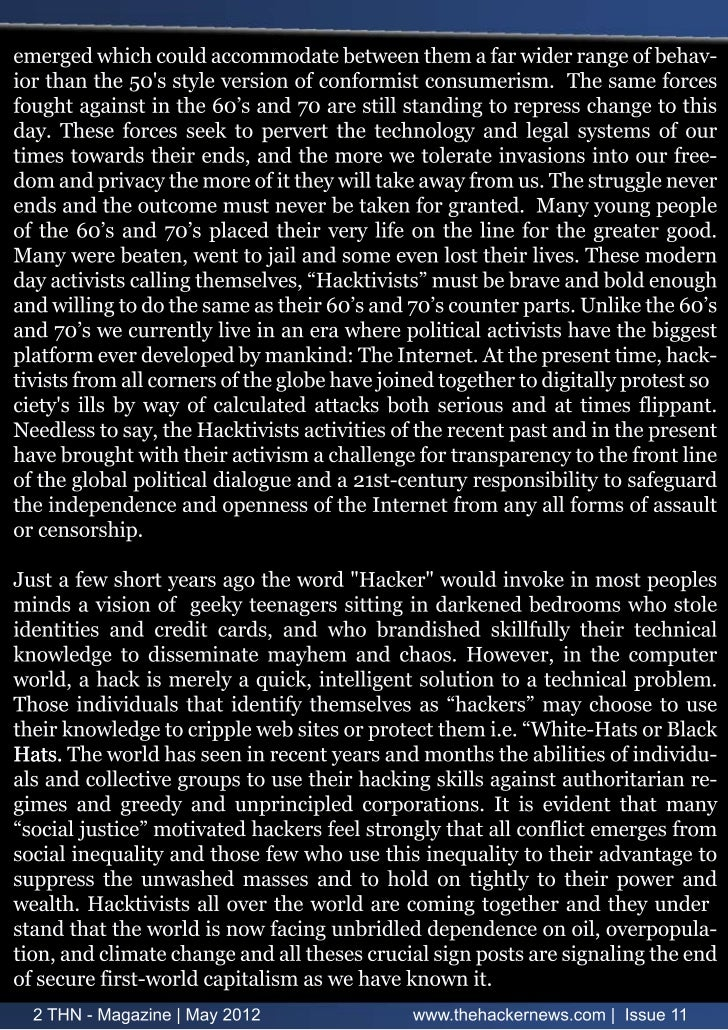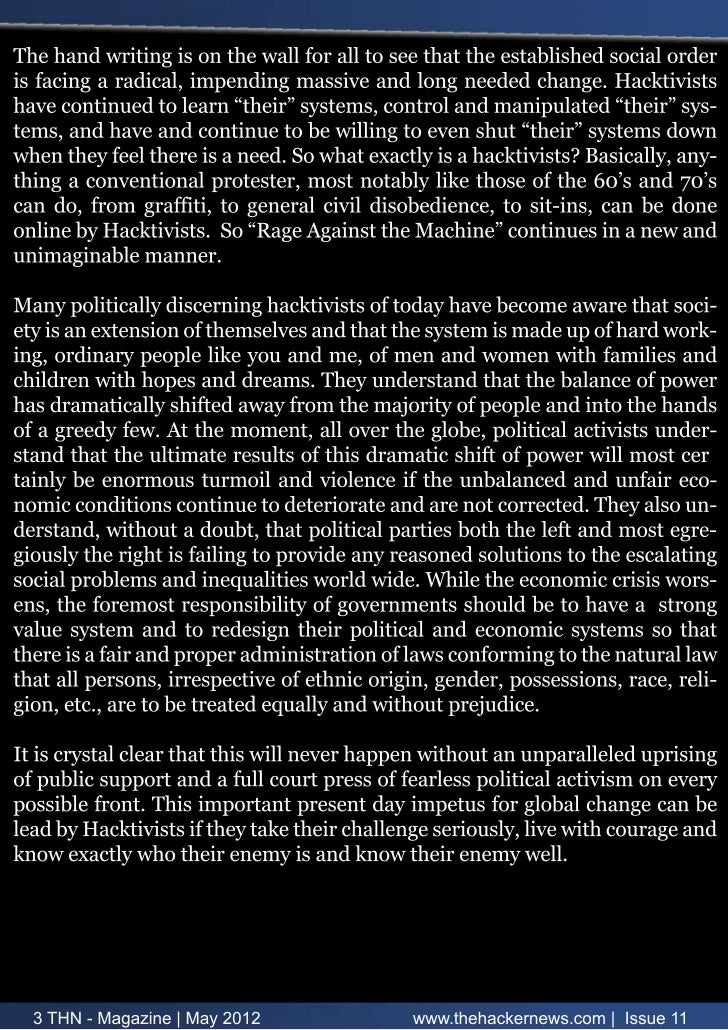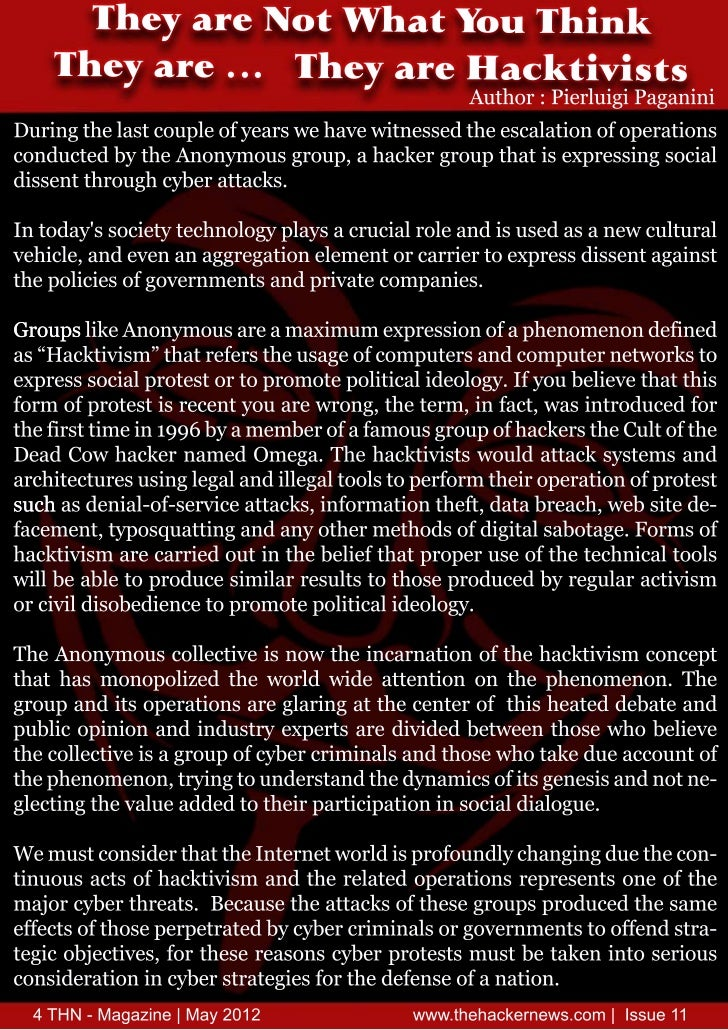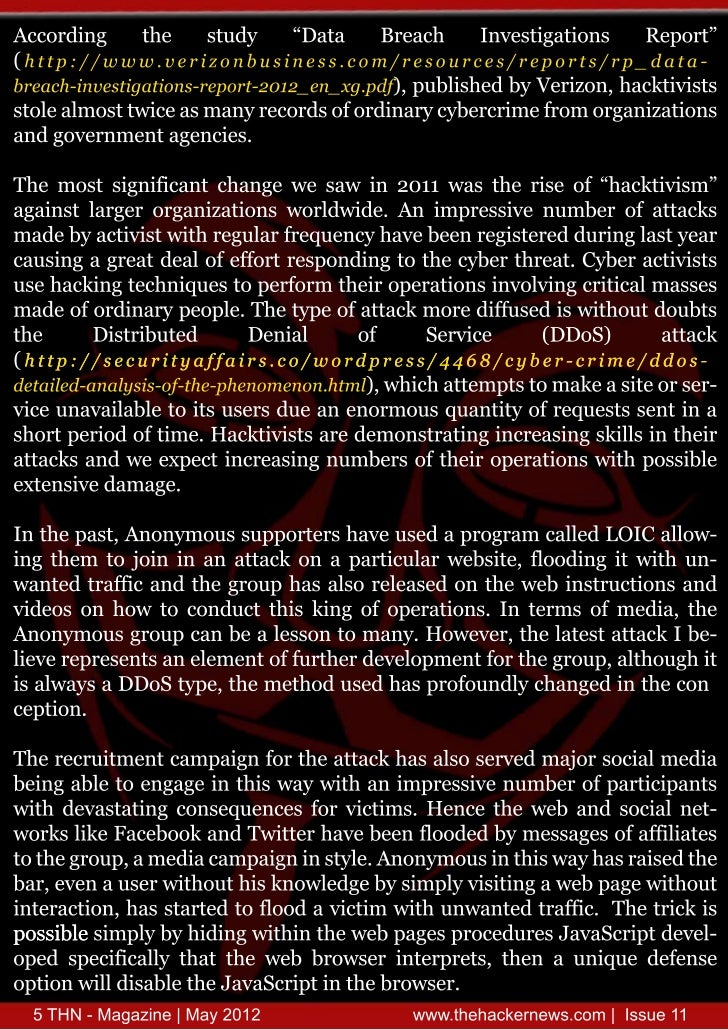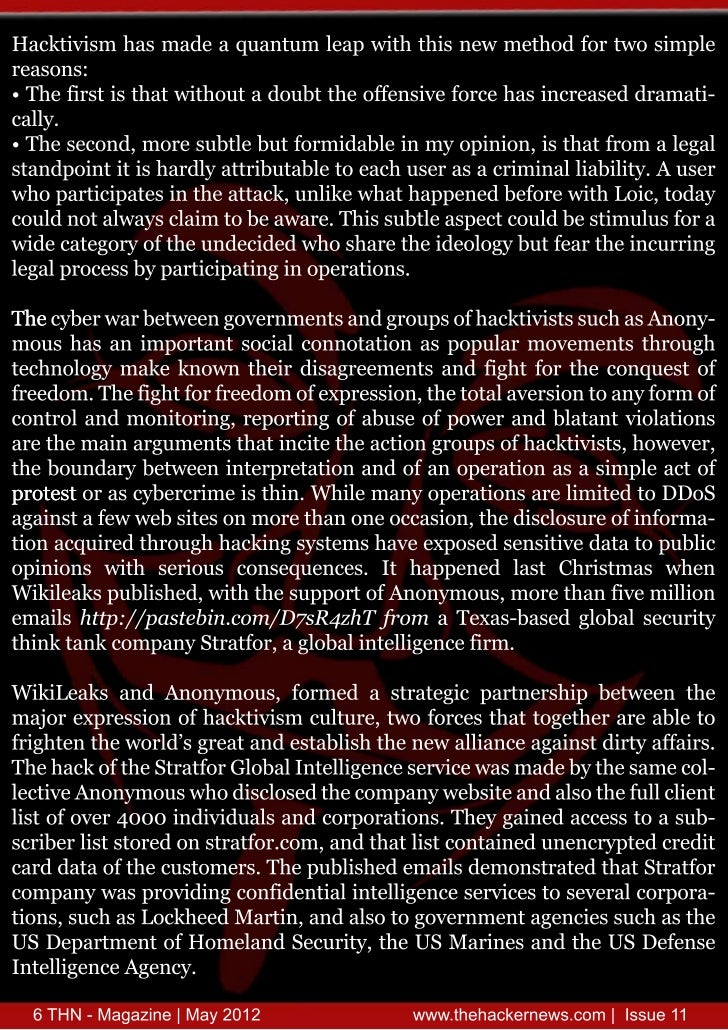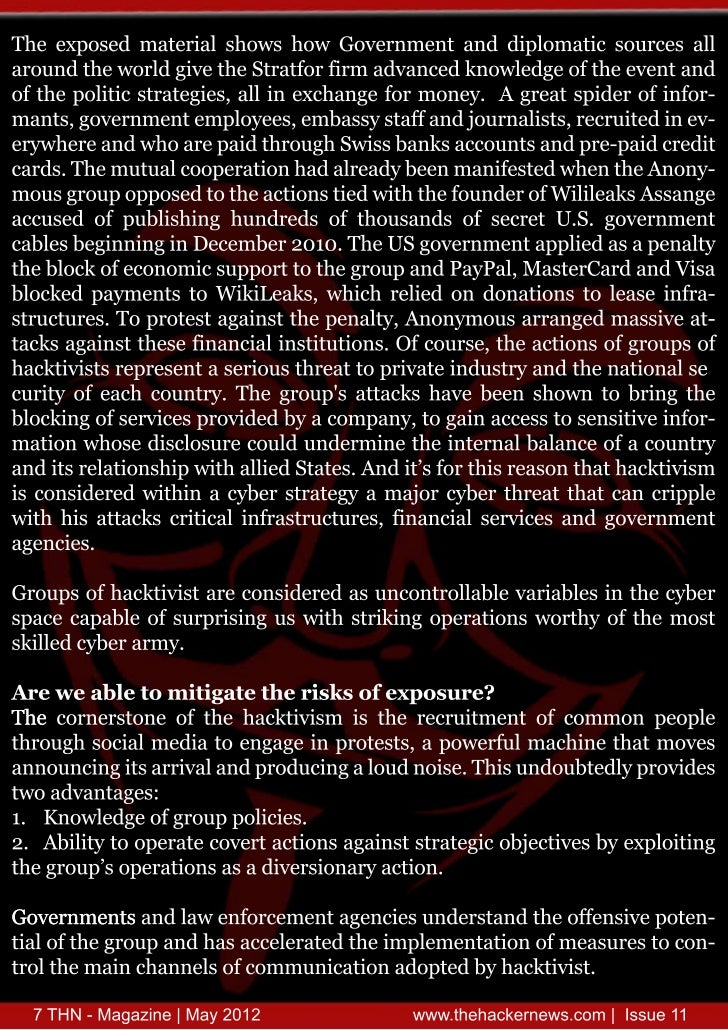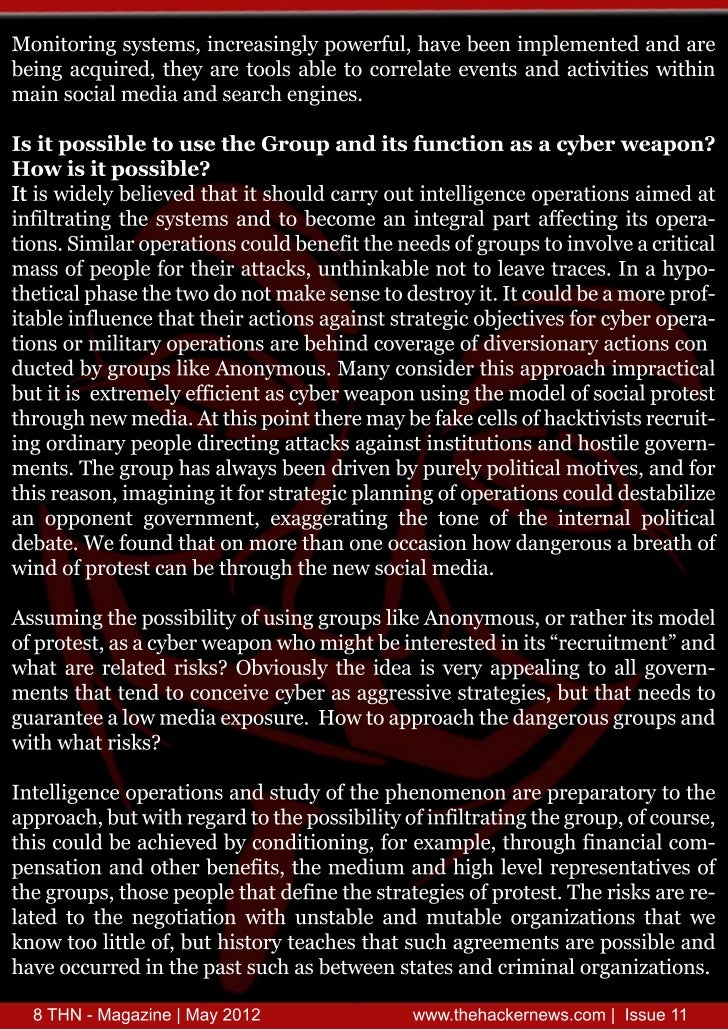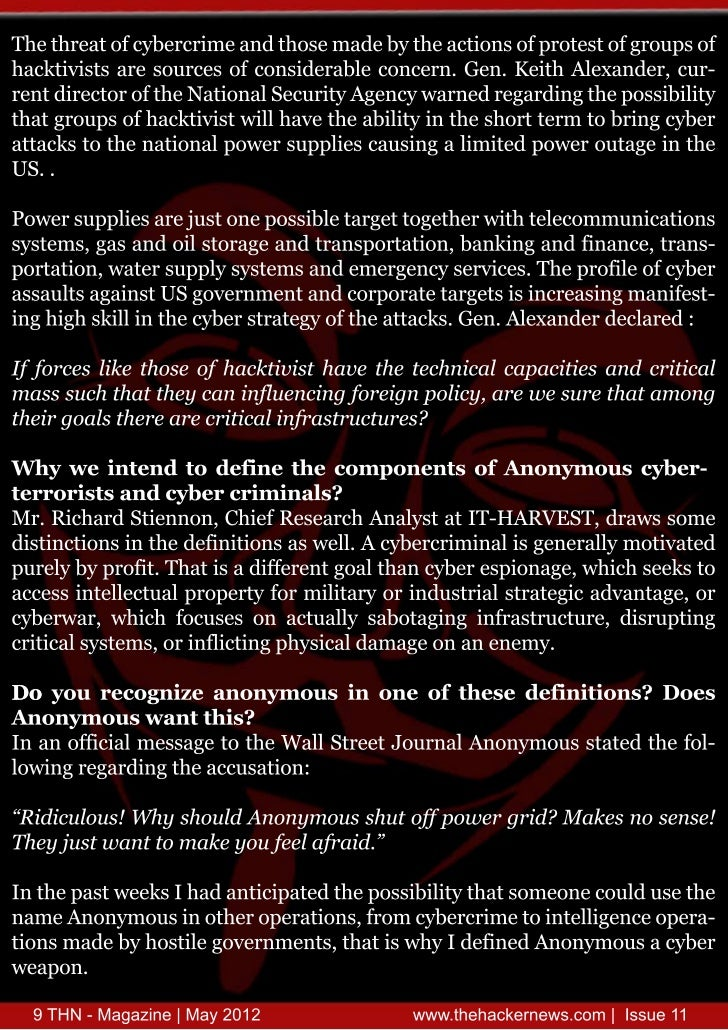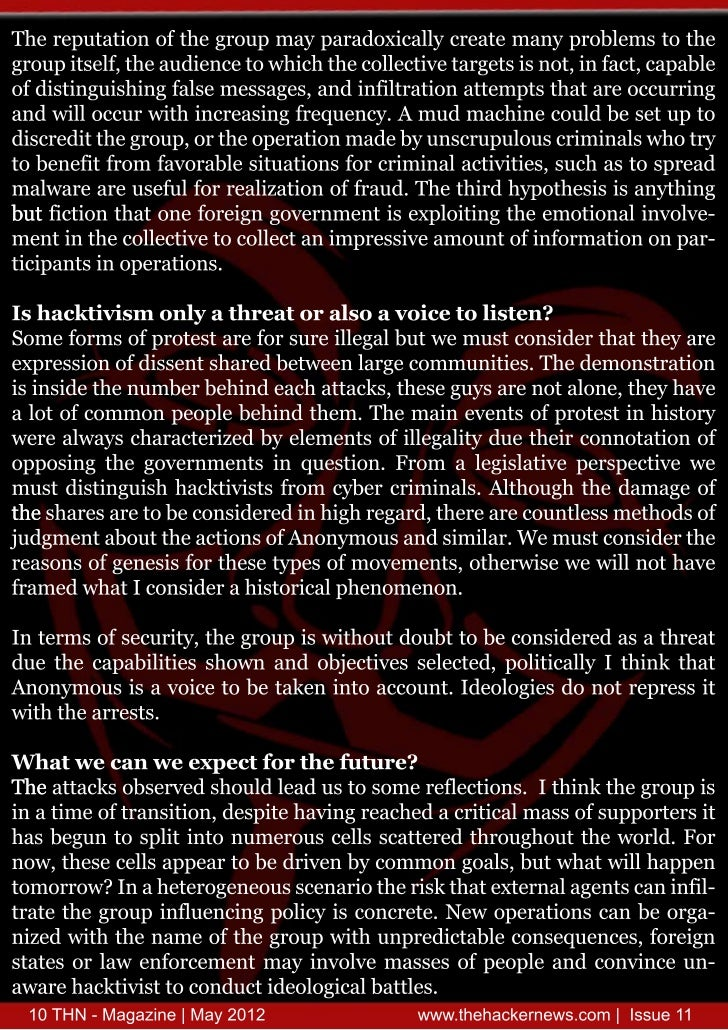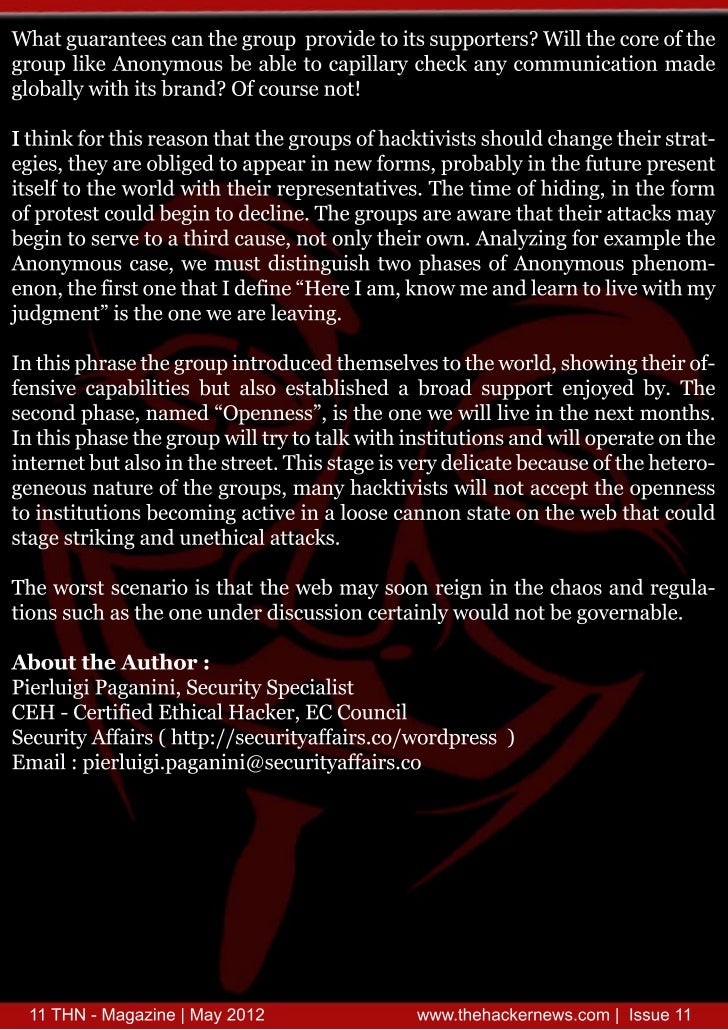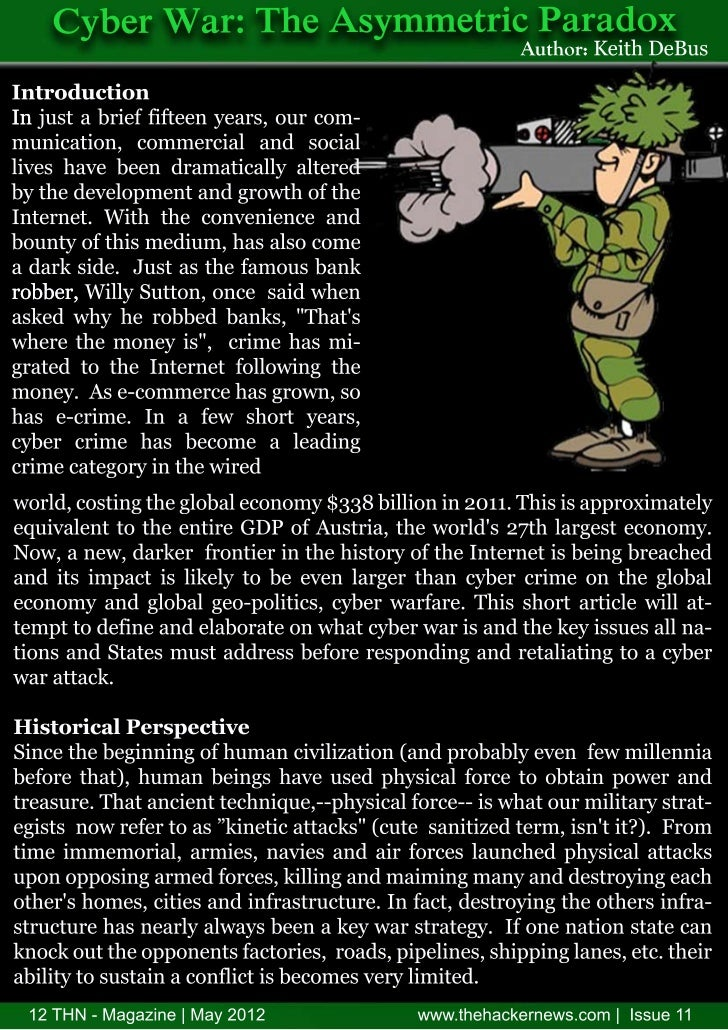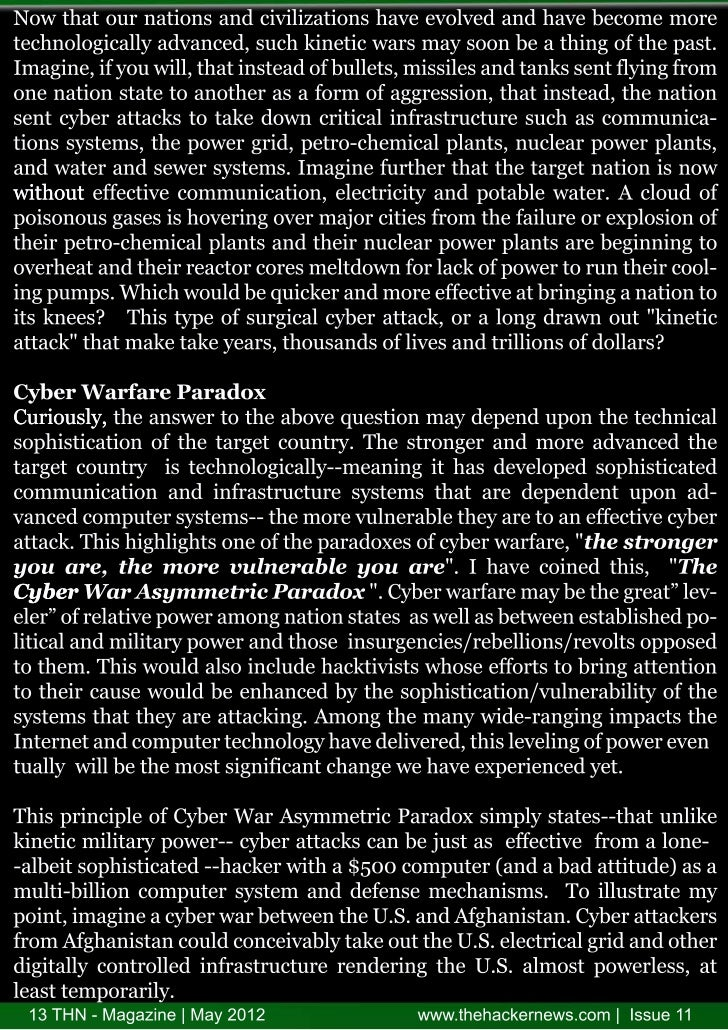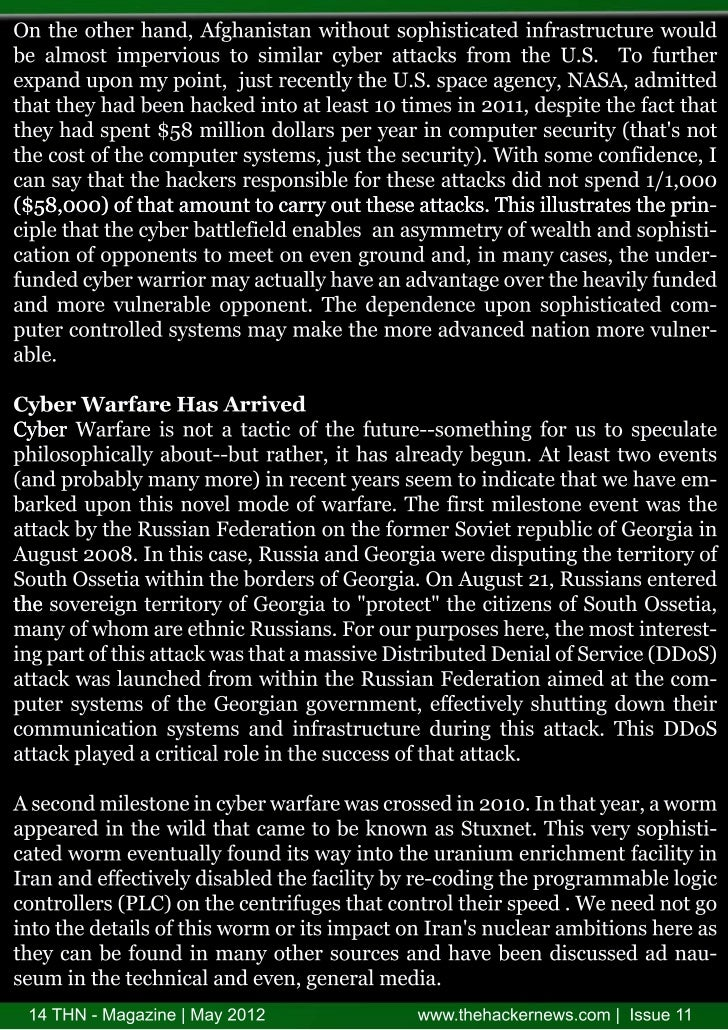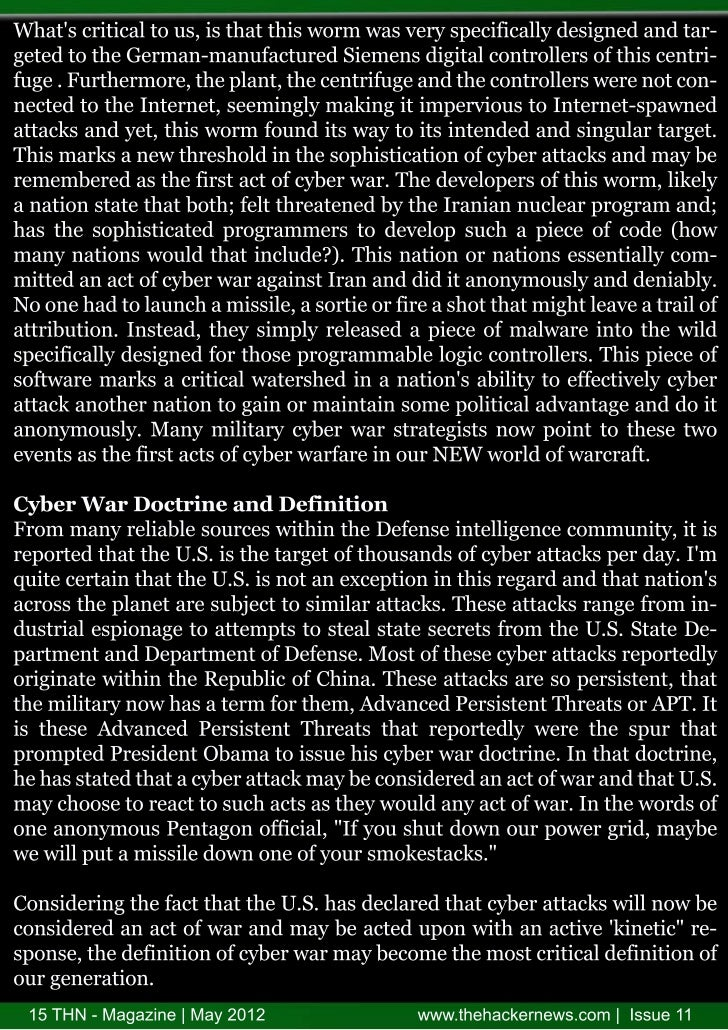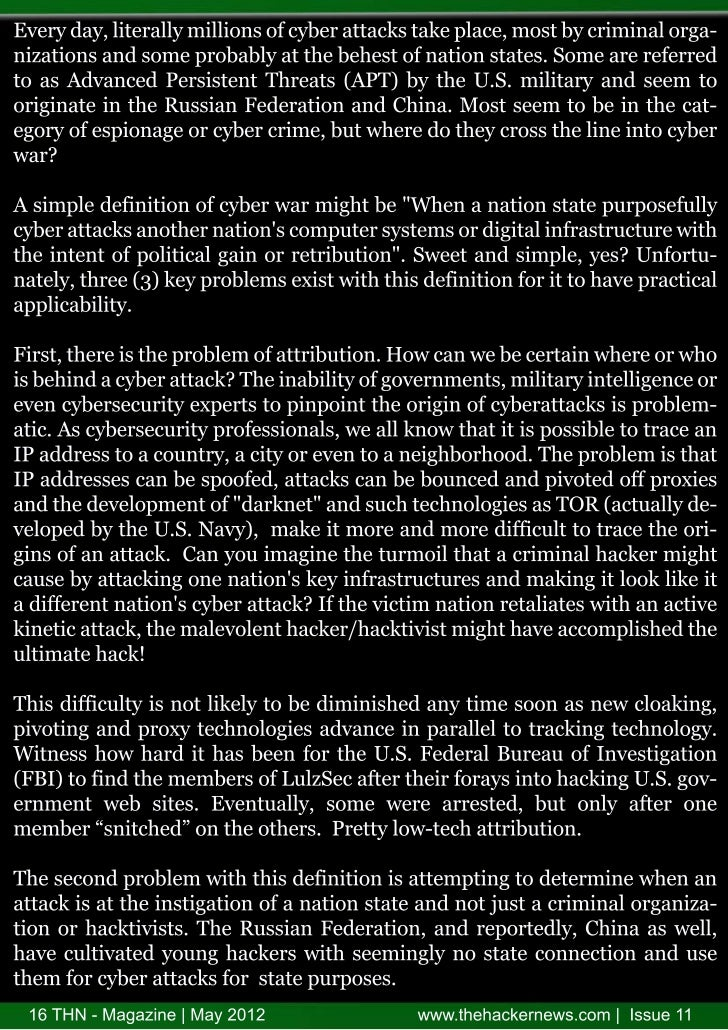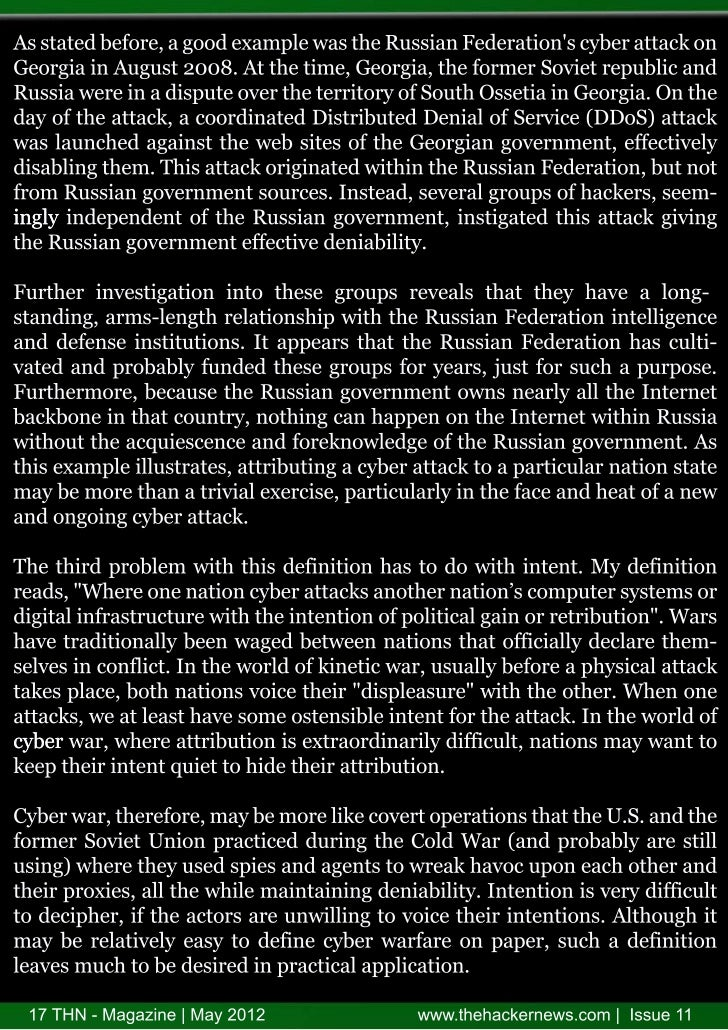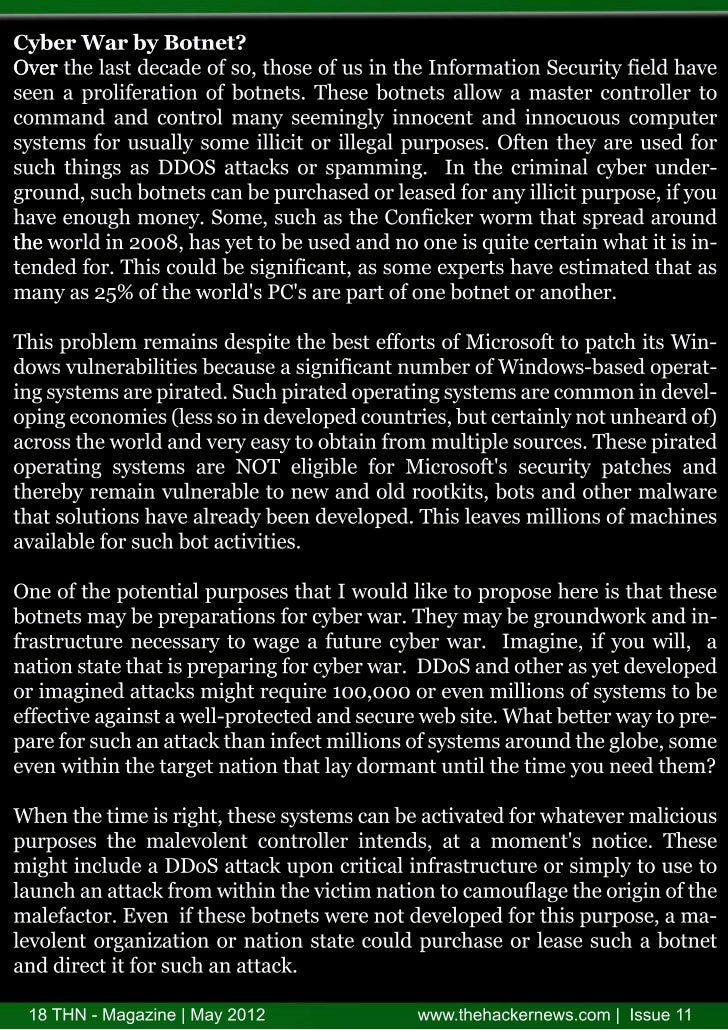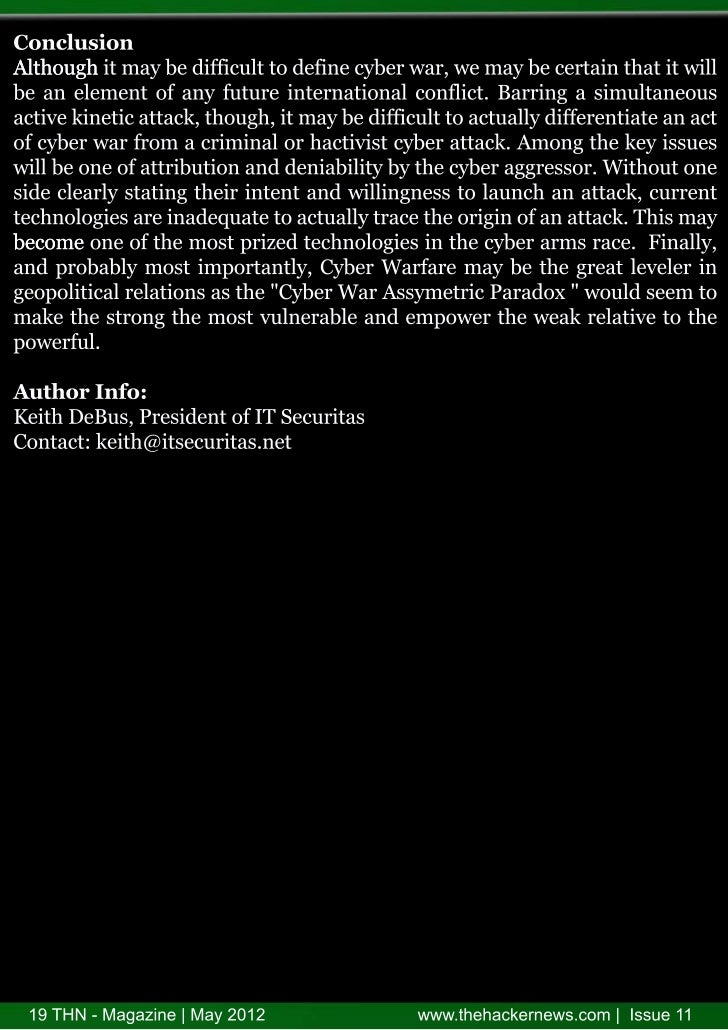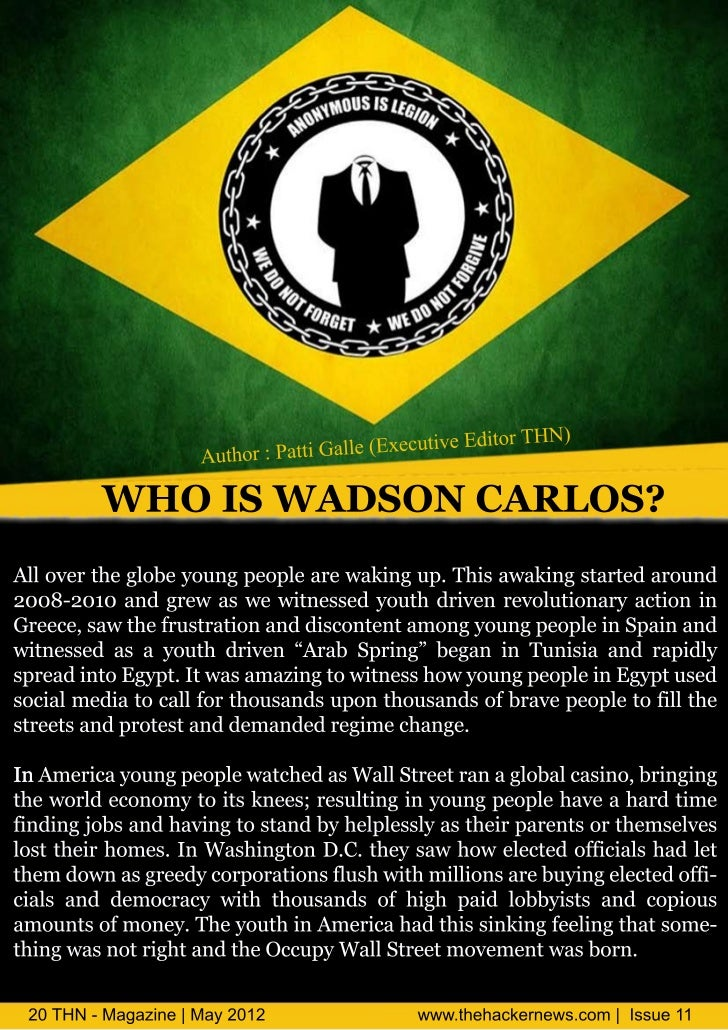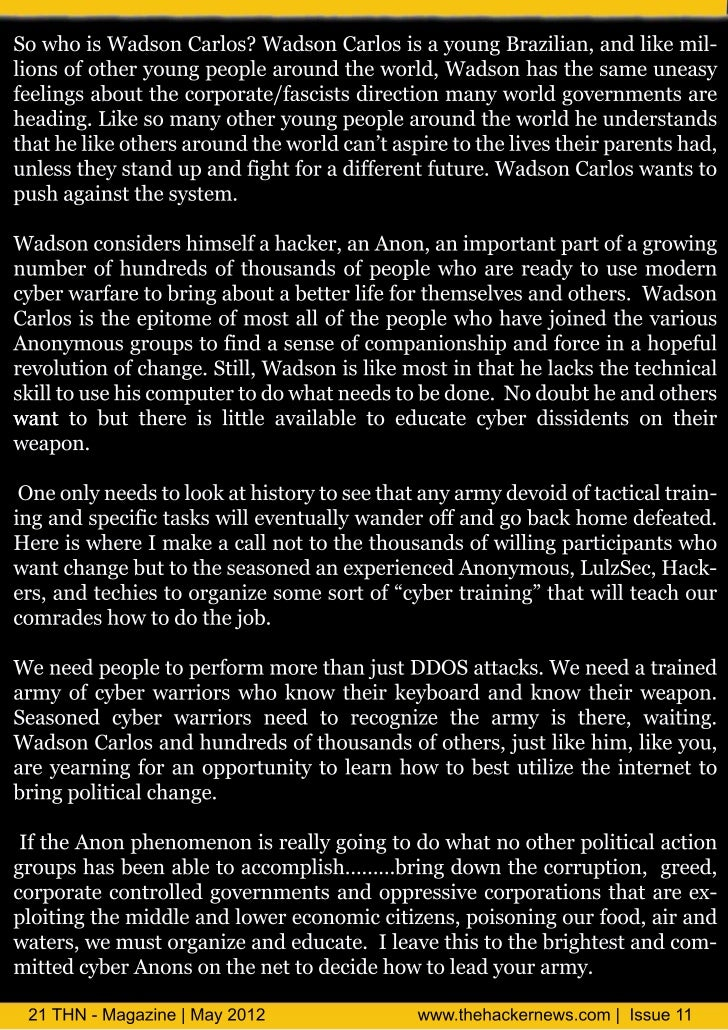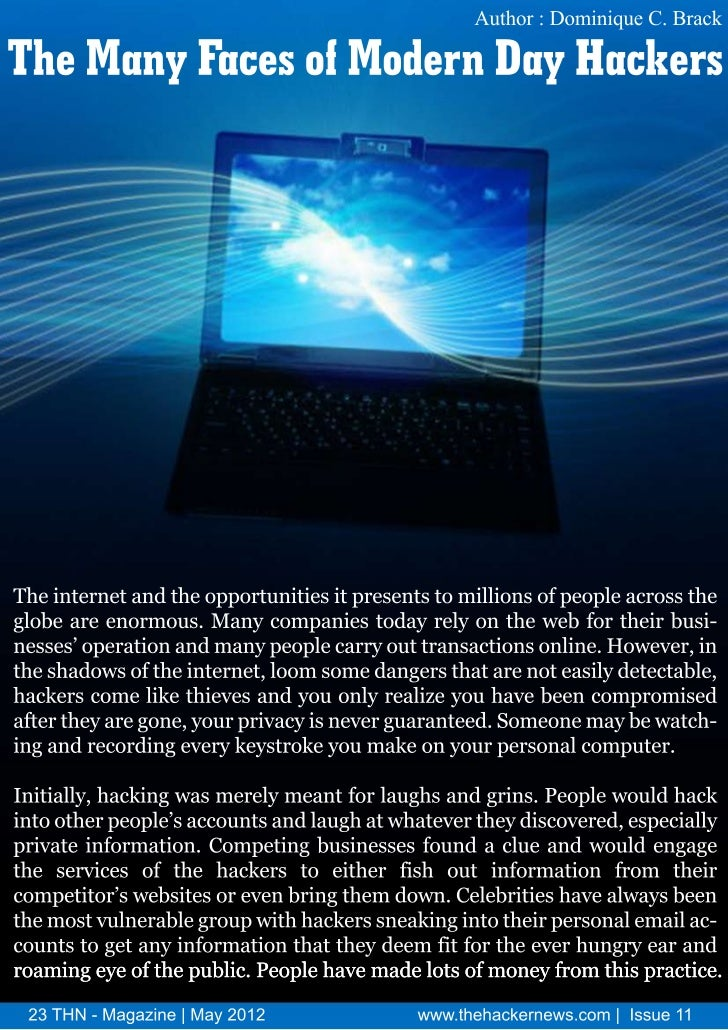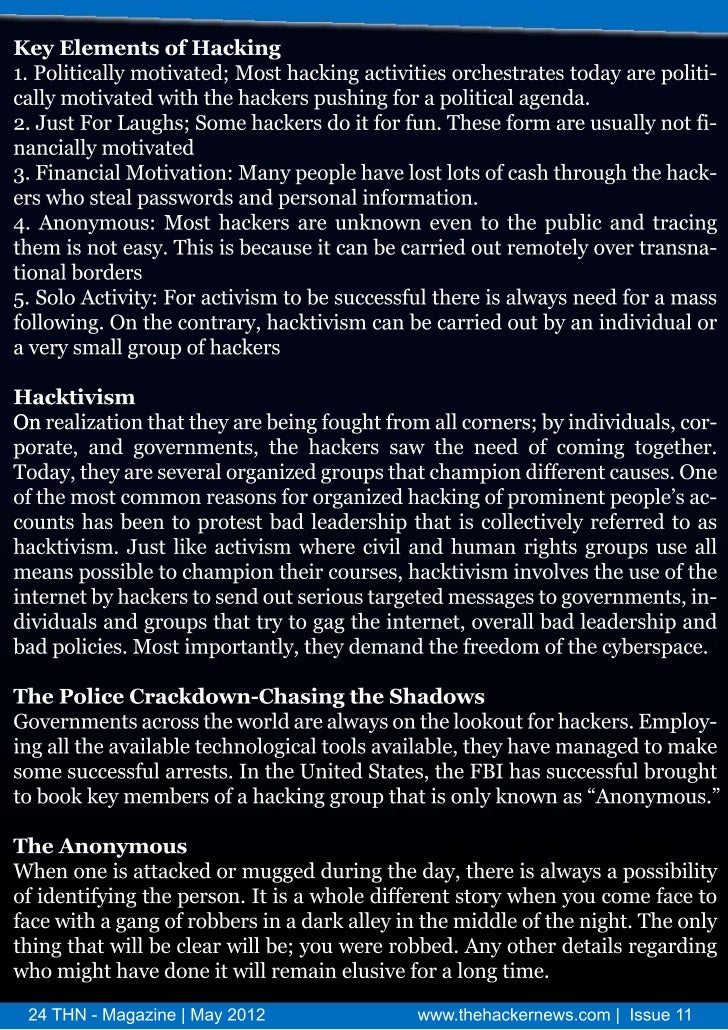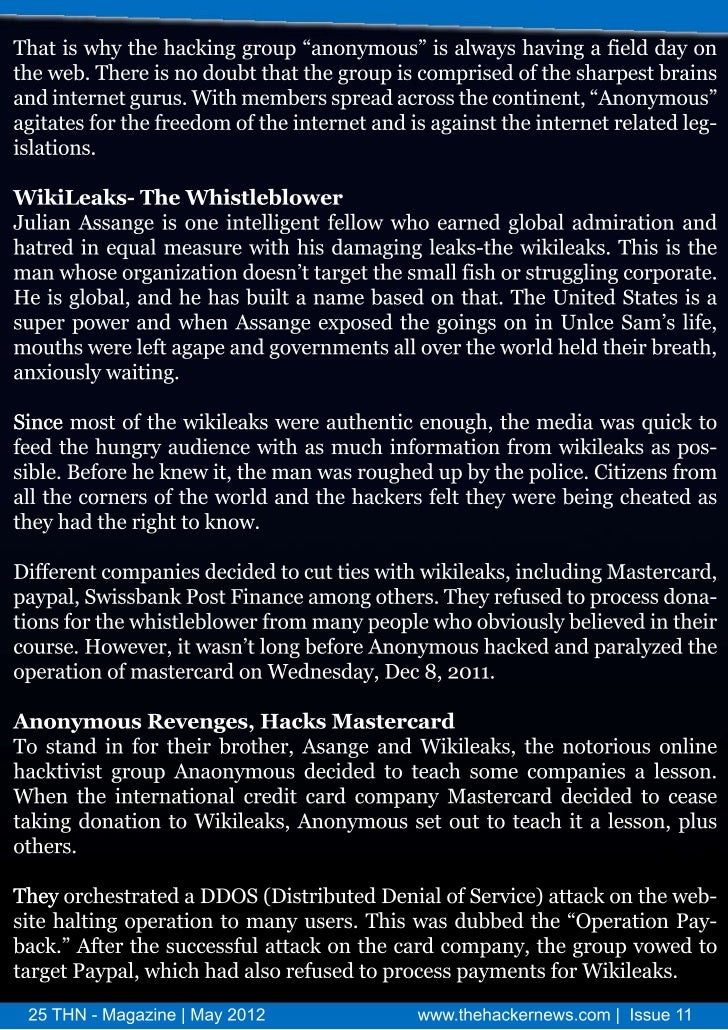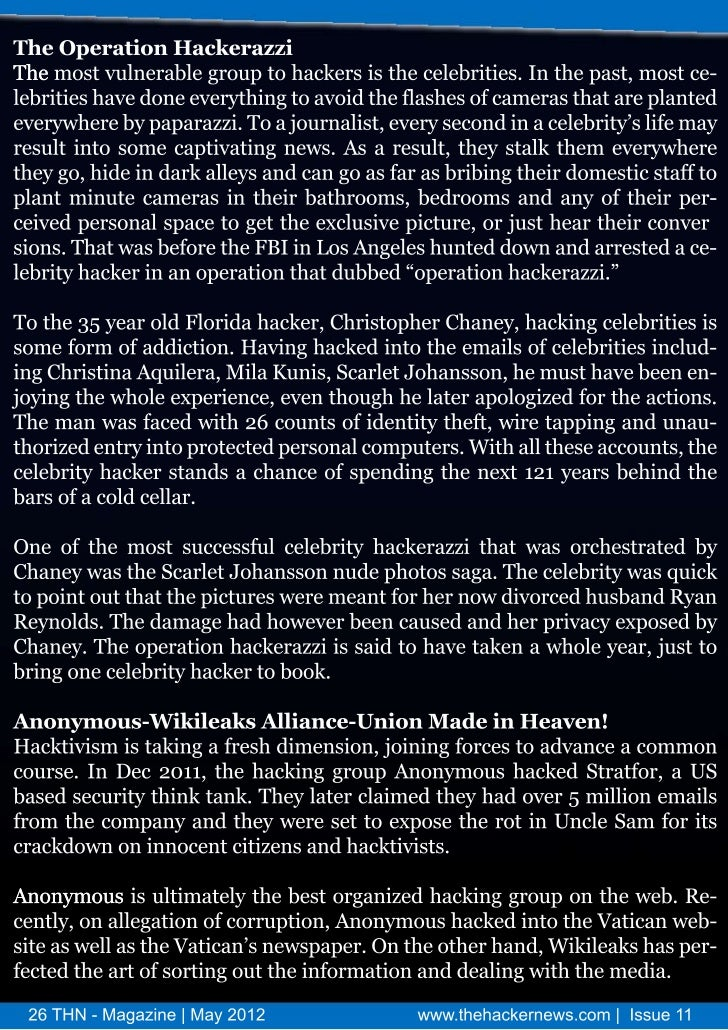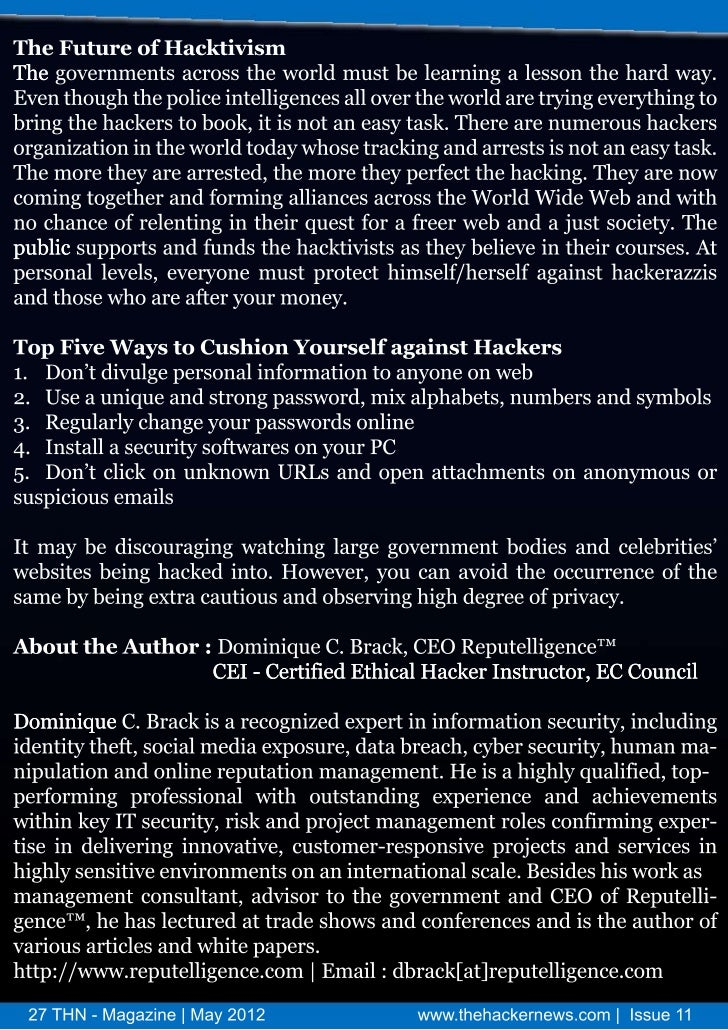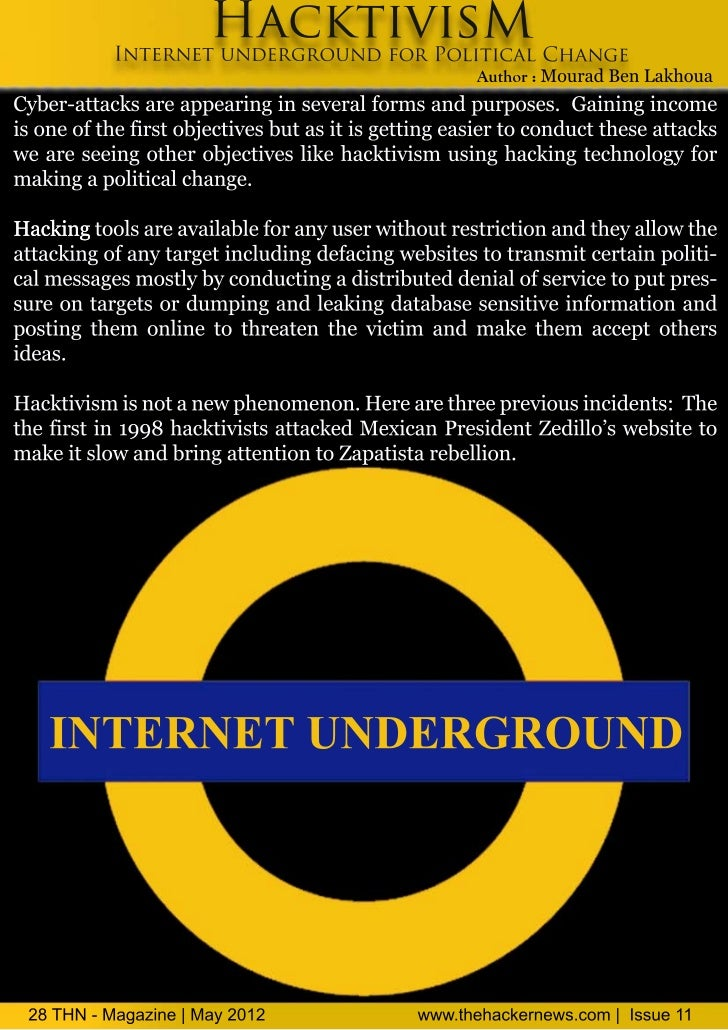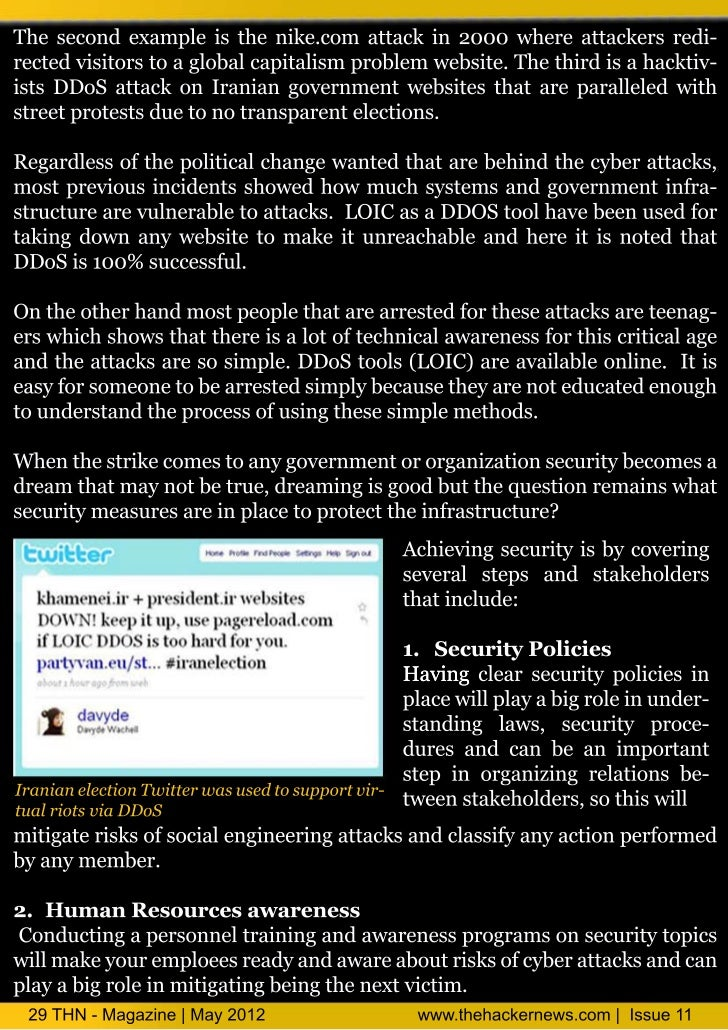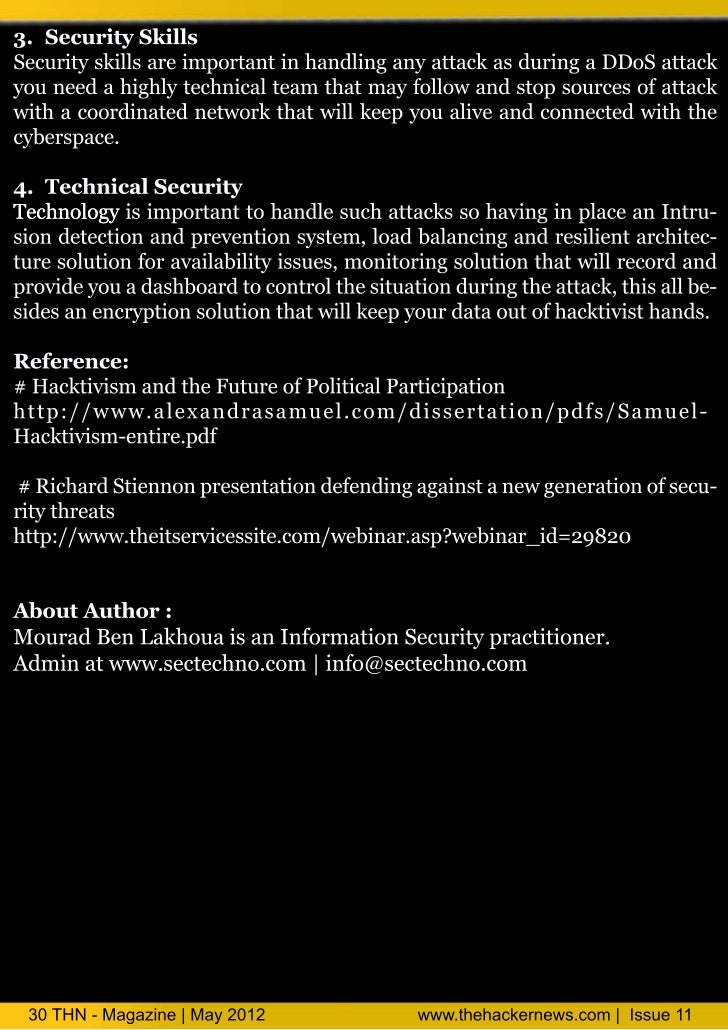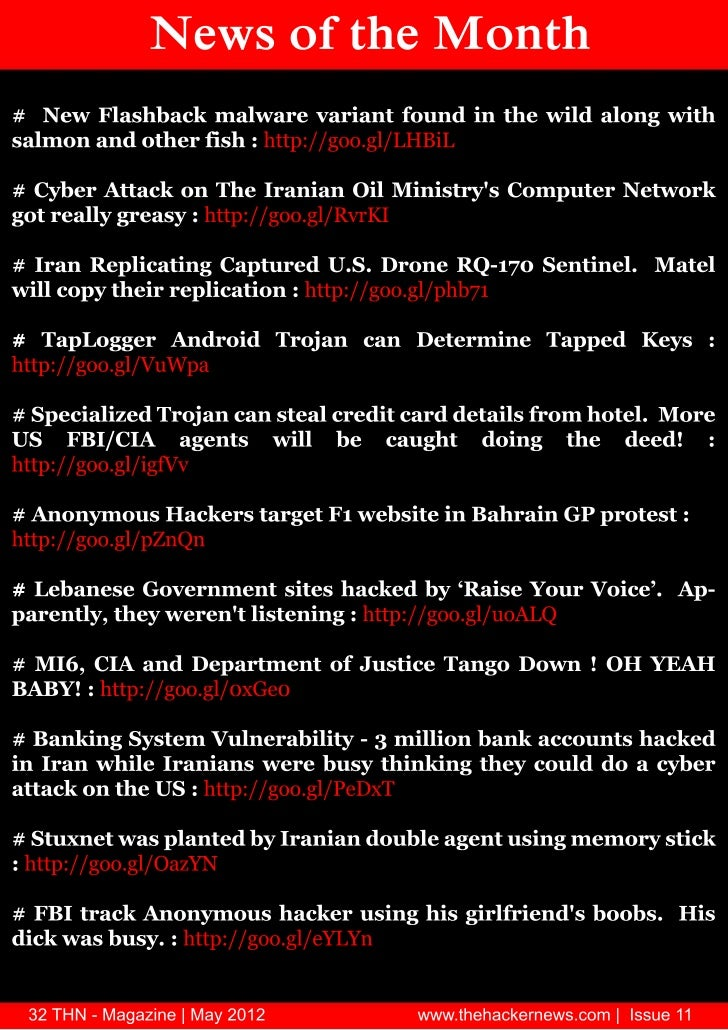Upcoming SlideShare
Loading in …5
×

# Hacktivism - The Hacker News Magazine - May 2012 Issue

Article from me „The Many Faces of Modern Day Hackers“ inside this issue. Welcome cyber space readers and internet junkies from around the world. May brings us into an in-depth look at our favorite topic: Hacktivism

• Full Name
Comment goes here.

Are you sure you want to Yes No
Your message goes here• Be the first to comment

• Be the first to like this

### Hacktivism - The Hacker News Magazine - May 2012 Issue

1. 1. T EH CKE E H A R N WS ,s Is e1 u1 02H C TVS May21 A KI M I
2. 2. E io i l d t raWe o cb rsaera esa d itre jn i f m ao n te l me y e p c ed r n nen t u ke r c s o ru d hw r . y r g u it a i-e t lo a o raoi tpc Haki ol Ma bi s sno n nd ph o k t u fv r eo i d n t : ct - vismO rer s l d rMo iK ma, u d r f h Hakr e s p n te u fal se e, ht u rf n e o T e ce N w o e sh e a odsus nwt a o ka te a ig f civ m a dw a imen i si i lo th menn o Hakt i n h tt a s c o h isfr oi y o a.o sc t td y eO r eua w i r, eui sei i Pelii aa iia dMo rd u rg l r es scr y p c lt i ug P gnn, n r t t as r uaB nL k o s bigu te p rp c v o ti mot neet ga d e a h ua r n s h i eset e n hs s itrs n n r i ito g t rv kn tpch u h po o ig o i.A e i rIrl e jydK i H. e u’at l o cb r a.Io n s dt , t y no e e h D B s r c n y e w r fu d o u t s iemye w a p d p nec e n a d or a h tks sno h w a’ sl rp e u i xi me t n w ry s e a e u it te h t f t sa dw ee o cb r a. n h r’ fy e w r sAs, o n u CB ak os necl n jbdsus g h tpc l D miq e rc d e a xe e to i si te o i o l c n .Y u eeui e i rP t G l ,r g y u o u s o jsw a a o y o r xct e dt , at a ebi s o t q et n ut h t n n - v o i l n imo s ed t lo l ente uue n d nt s o ru p ks tu- u n e so o k i i h ftr a d o ’ s u fn o e a cr k mirn n w .et e sT a k fr o ra hu ra esi a d h n so h s w o o t b ti h n so y u fi fled rhp n ta k t toe h cnr uen t is ma yma y aso n , n w y!Mo iKu r h t ma ,E i ri -h e, dt - c if o nT eHa k r ws h c e Ne T N- g z e| y 0 2 H Ma a i Ma 2 1 n w wte a k re s o |I u 1 w . h cen w . m s e 1 h c s
3. 3. T be f o tns a lo C ne t1 T e w knn o Haki s. h A a e ig f ct i ) vm Mo iK ma (dtr nc i , HN ht u rE i - -he T ) oi f2) h y r No Wh t o T ikT e ae h y r Hakii s .T e ae t a Y u hn h y r …T e ae ct s vt Pelii aa ii i ug P gnn r3)C b r rT e smmer P rd x . y e Wa: h A y t c aa o i Ki D Bs eh eu t4) .WHOI WA S NC R O ? S DO ALS P t G l (xct e dtr HN at a e E eui E i T ) i l v o5 T e n F cs f d r D y ces . h Ma y ae o Mo en a Hakr ) D miiu C B ak o nq e . rc6) ct i :nen t n ego n fr oicl h n e .Haki s Itre u d rru d o P li C a g vm ta Mo rdB nL k o a ua e a h u7 Hakn N w o te nh . cig e s fh Mo t ) T E i r l tf HN dt i Saf oa
4. 4. Te wk h A ae Auh r M to : oht u a iK m r nn o Ha ig f c ( dtrn Ei -- o i ci , he T N) f H kii t s vmHaki s ( a o iaino t o o moe w rs r rh me it o e ct i i cmbn t vm s o fw (r r) od o mop e sno nn ww r ( p r neu f ak n at i Haki s ite s o cm- e od a ot ta o h c a d c v m) ct i sh ue fo ma is vmp tr a dcmp trn t ok a amen o poet opo t p li l ues n o ue ew rs s a s f rts t rmoe oictae d. h tr w si t on di 19 b a mb r fh C lo te ed n sT e em a fs cie n 9 6 y me e o te ut fh D a rC w h ce cl c v n me O g. f akn a "l a ybekn it o akr ol t e a d mea I h cig s ie l ra ig no ei lg lcmp tr"s su d te h ct i cudb d f e a " e o v l t o ues iasme ,h n aki s o l e ei d s t n n i e vm n h onue fea a d o iea dgtlo li p rut f oicl n s ~Wiie s o l l n /rl gl ii tosn usio p li e d" g l a ta kp -daT e re n ylp da i h Fe E cc e i , oS vrl ea e atrh mot xe s e nia mo e n i A r a hs eea d cd s f te setni a t r vme tn mei n i e v w c -tr,h poeto te 9 0 a d 9 0 o e o p s in o mei n n ov-oyte rts fh 16 s n 17 s vr p oio t A r a iv l s t c eme tn h Ven m Wa,h p li l att o td y o t u t sac fr n i te i a t rte oic l s e fo a cni e o erh o tay u ncet ew y t “aeo ”aa s sc l nut e. h mots nf a t rai as o rg n gi t oi ijs cs T e s i icn v n a i g il snta w td y a l r f m te oi mo e ns fh 6 s n 7 ’e o h t e o a cn e n r h sc l vme to te 0 a d 0s s a o aita tig cudb c a gdie o g p o lra z ih so a p n J ssh thn s o l e h n e f n u h epe elet a t h p e .ut il etd y te6 ’a d7 ’makdada t si i tikn ; s dai o a,h 0s n 0s re k rmai hf n hn ig a a r c tmairt curdi te utrlo snu,n a ei o sbutrs t i ocre n h cl a cne ssa d sr s fu cl e cf u e u 1T N- g z e| y 0 2 H Ma a i Ma 2 1 n w wte a k re s o |I u 1 w . h cen w . m s e 1 h c s
5. 5. e re w i cud co megd hc o l acmmo ae ew e te a a wd ra g o b h v h d t b t en h m fr ie rn e f e a-ir h nte 0s tl vrino cnomi cnu r m.T e a freo ta h 5 s e es y o f o fr s o smei t s h sme ocsfu h aa s i te 0s n 7 ae tltn igt rpes h n e otio g t gi tn h 6 ’a d 0 r s ls dn o e rs c a g t hs n i ad y T eefre se t p ret h tc n lg a dl a ss mso o r a. h s ocs ek o evr te eh oo y n e l yt g e fut s o ad te e d, n temoew tl aeivs n it o r rei tw rs h i n sa d h me r r e oe t n ai s no u f - r o ed m a d r ay h moe fth y i tk a a f m u. h s u g n vr o n pi c te r o ite wl a e w yr v l o sT e t gl ee r ee d a dte ucme s n vr e a e fr rne .Ma y o n p o l n s n h ot o mut ee b tkn o ga td n y u g epeo te6 ’ a d7 ’ pae te vr leo tel efr h getr o d f h 0s n 0s l d h i ey i n h i o te rae g o . c r f nMa y ee etn w n t j l n sme vnls te l e. h s mo en n w r b ae , e to a a d o ee oth i i sT ee d r i rvd y c v tcln te e e,Haki s ” sb bae n b l e o g a at i s a ig h msl s“ ct i s mut e rv a d od n u h is l v vta d iig o o h sme sh i6 ’a d 0s o ne p r . nie h 6 ’ n wln t d te a a te 0s n 7 ’cu tr at U l te 0s l r s ka d7 ’w cre t l e na ea h r p li l c v t h v te igs n 0s e urnl i i n r w ee oic at i s ae h bget yv ta ispafr ee d vlp d y n id T e nen tA te rsn t , ak l om vr ee e b ma kn : h Itre. th pee ti h c- t o met i srm a cres fh g b h v jie tgte t dgtl poetois f v t o l on r o te l e aeon d o eh ro ii l rtss l o ayc t isb w yo cl l e atcsb t sr u a da t sfp a t i y l y a f a ua d t k oh ei s n t i e s l c t a o me l p n. iN e l so a,h Haki s at ie o te ee t at n i te rsn ede t syte ct i s c v i fh rcn p sa d n h pee t s v t it sh v bo g t i te at i a h l n eo t np rn y o h f n l e ae ru h wt h i c v m c a e g frr sae c t ter ti h r is l a o no te lb l oicl i o u a da 1t e tr rso s it t sfg ad fh g a p li da g e n 2s cnuy ep ni ly o aeu r o ta l - bite n e e d n e n o e n s o te nen trm a y lfr o asuth id p n e c a d p n es fh Itre f o n a oms f sa l lo cnosi. r e srhpJ s afw sot er aote od" ce" o l iv k i mot epe ut e h r yas g h w r Hakr w ud n o e n s p o l smid av ino geyte aes iigi d re e b do ms h s l n s io f ek en gr s t n akn d e ro w o t e s tn oie ti a d ce i crs a d w o ba dse sifl te tc ncld nie n rdt ad, n h rn i d klul h i eh i ts h l y r ak o l g t dse n t mah m a dc a s Ho ee,ntecmp tr n we e o i miae y e n h o. w vr i h o ue d sw r , h c i mee aq i ,ne ie t ouint atc ncl rbe ol a ak s rl d y uc itlgn slt o eh i po l k l o a m.T oeidv u l ta ie tyte e e a “akr” yc o s t ue h s n i d a h td ni h msl s s h ces ma h oe o s i s f vte k o l g t cipe e s e o poeth m i .Wht- to Bakh i n we e o r lw b i s r rtcte . “ i Has r l r d p t e e cHasT e ol h s eni rcn yas n mo tsh a it s fn i d - t h w r a se n ee t er a d nh te bli o idv u . d ie ia a dcl c v go p t ue h ih cigsis gis a toi r nr- l n ol t e ru s o s te akn kl aa t uh r ai e s ei r l n t agme a dged a du pic l croain. t seie t h t n i s n re y n n r i e op rt s I i v n ta ma y npd o d“oi js c” t ae h ceselt n l ta a cnl t megsrm sc lut e moi td akr fe s o g h t l o fc e re f a i v r y l i osc ln q a t a dtoe e w oue hsn q a t t te a vnae o oi ie u ly n h s fw h s tiie u ly o h i d a tg t a i i rsp rs teu w se massa dt h l o t hl t te p w ra d u pes h n ah d se n o od n i t o h i o e n g y rw a h Haki s a o e tew r aecmigtgte a dte u d r el . ct i s l vr h ol r o n o eh r n h y n e t vt l ds n ta te ol in wfc g n r l d p n e c o olo ep p l t d h th w r s o ai u bi e e e d n e n i vro ua a d n dd , -t n a d l t c a g a d l h ss rc lin otae in l g h e di ,n c mae h n e n a tee cui s p s r s a n te n o i l a g s g io scr fs w r cptlm a w h v k o ni feue i t ol a i i s e ae n w t r- d as . 2T N- g z e| y 0 2 H Ma a i Ma 2 1 n w wte a k re s o |I u 1 w . h cen w . m s e 1 h c s
6. 6. T e a dw in io te a fr lt se h th etbi e sc l re h h n r ig s n h w l o a o e ta te s lh d oi od r t l l a s ai fc gardcli e dn mas ea dln n e e c a g. ct i ss ain a i ,mp n ig si n o g ed d h n e Haki s a v vth v cniu dt l r “ e ” ytmscnrln ma iuae “ e ” y- ae o t e o e n t i ss n a hr e ,o t a d np l d t i ss o t hrtmsa dh v a dcniu t b wln t ee su “ e ” ytms o ne , n ae n o t e o e iig o vn h tt i ss n l hr e dww e te fetees n e . o h t xc ys h ct i s B s a ya y h n h y elh r ia ed S w a eat ia aki s ? ai l ,n - l vt cltigacn e t n l rts rmot oa l l etoeo te6 ’a d7 ’hn o vni a poet , s n tby i h s fh 0s n 0s o e kcnd ,rm gafit gn rl i l i b de c,os - s cnb d n a of o rft o e ea c ids e i e t ii , a e o e i, v o n tno l e y ct i s S “ ae giste c ie cniu sna e a d ni b Haki s . o R g A a th Mahn ” o t e i n w n n vt n nu i gn be n e. nmaia lma n rMa y oicl dsenn h ct i s fo a h v b cme w r ta sc n p li l i rig aki s o td y ae eo a ae h toi tay c vt -eys n xe s n fh msl s n ta te ytm ima e p f ad ok t ia etni o te e e a d h th ss o v e s d u o h r w r-ig odn r p o l l e o a dme o me a dw me wt fmie a dn , riay epe i y u n k , f n n o n i a ls n h ic i rnwt h p s n demsT e u d rtn ta te a n e f o e hl e i o e a d ra . h y n es d h th b l c o p w r d h a ah s rmai l sie a a f m te jr y f epe n it te a d a da t a y hf d w y r h ma i o p o la d no h h n s cl t o oto aged fw A te me ta o e te lb , oicl c v t u d r f re y e . th mo n, l vr h g e p li at i s n e- l o ta iss n ta te lmae eut o tida t si o p w r i mot e t d h th ut t rsl fhs rmai hf f o e wl s cr a i s c t ltil b e omo s umol n v l c iteu b l cda du fi eoa y e n r u tr ia d i e e fh n a n e n na c- n on a rn micn io s o t u t d tr rt a d r n torc d T e a o n o c o dt n cni e o eei ae n ae o cret . h y l u - i n o e sd rtn , i o t d u tta p li l at s ohte et n mot ge es d wt u a o b,h t oic p r e b t h l a d s er- a h ta i fgo s te ih ifin t po ie n rao e slt n t te sa t g iul h r tsa ig o rv a y esn d oui s o h ecl i y g l d o ansc l rbe a die u li w r wd . i te cn micis os oi po l a ms n n q a t s ol ieWhl h eo o c r iw r- ie d e se ste oe s rso s it o g vrme t so l b t h v a s o g n,h frmot ep ni ly f o en ns h ud e o ae t n bi rvless m a dt rd s nte p li l n eo o css mss ta au yt e n o e ei h i oic a d cn mi yt g r ta e o httees fia d rp r d ns ain fa s o fr n t te aualh r iaa n po e a mii rt o l cnomig o h n trl w r t o w ata a p ro si ep c v o eh i oii, e d rp ses n,ae rlh t l esn,r set e f tnc r n gn e, ossi src,e - l r i g o igo ,t ,r t b t ae e u l a dwto t rjdc. in ec ae o e r td q a y n i u pe i . e l h u eIicytll rh ths i n vr a p nwto t nu p rl l u r igts rs c a ta tiwl ee h p e i u a n aa e d pin a e l h le so p bisp ot n a ulo rpes fer s p li l c v m o eey f u l u p ra d flcut rs o fal s oic at i n vr c e ta isp sil f n. hsmp r n pee t a i eu fr lb l h n e a b os e r tT ii ot t rsn d y mp ts o g a c a g cn e b o a ol d y ct i sfh y a e h ic a e g sr ul l e i cuae ne b Haki s ite tk te h l n e ei s , v wt o rg a d a vt r l o yi hk o eat w o h ie e ia dk o te e e w l n w xc y h te n mys n n w h i n my e . l r r l 3T N- g z e| y 0 2 H Ma a i Ma 2 1 n w wte a k re s o |I u 1 w . h cen w . m s e 1 h c s
7. 7. T e a e o Wh t o T i k h y r N t a Y u hn T e ae h y r … T e a e c tvss h y r Ha k iit A to : i lii aa ii uh r Pe ug P gnn rD r g h l to pe f er w h v wtesd h ecl ino o eain ui tea cu lo yas e ae i se te sa t n s n a o f p rt s ocn utdb te n n mo s ru , h ce go pta iepes g oi odc e y h A o y u go p a akr ru h ts x rsi sc l n adse tho g cb r t cs i n tru h y e at k. s aI td y sc t tc n lg pas cui rla diue a a e cl rln o a oi y eh oo y l a rc loe n s sd s n w ut a s e y a uvhc ,n ee a ageaine me t r ar ro x rs dse t gis e i ea d vn n grgt l n o cri t epes i n aa t l o e e s nte oi e o g vrme ta dpi t cmp ne.h p l i f o en ns n r ae o a i cs v sG o p l e n n mo s r a x m epes n f p e o n n eie ru si A o y u ae mai k mu x rsi o a h n me o d f d o na “ ct i ta rfr te sg o cmp tr a d o ue n t ok t s Haki s h teesh uae fo ues n cmp tr ew rso v m”epes oi poet ro rmoe oicld oo yIy ub l v ta ti x rs sc l rtso t po t p li ielg.f o e ee h ths a ta ifr o poets ee t o ae rn ,h tr i fc w snrd cdfrom f rts ircn y u r w o g te em,n at a it ue o , otei ti i 19 b a mb r f fmo s ru o h cesh C lo teh fst n 9 6 y me e o aa u go p f akr te ut fh r meD a C w h ce n me O g. h h ct i s o l atc ss ms n ed o akr a d mea T e aki s w ud t k yt a d vt a eac i c rs s geaa d l glo lt p r r te o eain f rts rht t e ui l ln ieatoso ef m h i p rt o poet eu n g l o r osc a d na o-ev e t csifr t ntetd t bec , e s e e uh s e i -f ri at k,nomai h f aa rah w b i d - l s c a o , tfcme ttp su tn a da y te meh d o dgtla oaeF r oae n,y oq at g n n oh r to s f ii sb tg. oms f i ah ct i ae ar do tnte e e ta po e ue fh tc nclo l aki s r cri u i h b l fh t rp r s o te eh i tos vm e i awlb a l t po ue i l rsl t toe rd cdb rg l at i i e be o rd c s a eut o h s po ue y eua c v m l mi r s r iso c idsb de c t po t p li ld oo y r i l i e i e o rmoe oic ielg. v o n taT e n n mo s ol t e s o te n an t no te aki s cn e t h A o y u cl c v i n w h icrai fh h ct i o cp ei o vmta h smo o oi dtew r wd atnin o tep e o n n T eht a n p le h ol ie t t z d e o n h h n me o . hgo pa di o eain ae l iga te e tr ftih ae d b t a d ru n t p rt s r g r s o a n th cne o hs etd e ae np bi o iina did s yep r aedv e b t entoew ob l v u l pno n n ut x et r i d d ew e h s h e ee c r s i ite ol t es go po cb r r n la dtoe h tk d e co n oh cl c v ia ru fy e ci a n h s w o a e u acu t f ei mi ste h n me o ,rig o n es n te y a c o i gn s a d o n -h p e o n n t n t u d rt d h d n mi ft e ei n n t e y a s s sg c n te a e d e t te p r c aini sc l i o u . l t g h vl a d d o h i at i t n oi da g e ei u r ip o a lWe scnie ta te nen t ol ipoo n lc a gn d eh cn muto s rh th Itrew r s rfu dy h n ig u te o - d dt u u at o h ct i a dterl e o eain rpee t o eo tei o s c f aki s n h e td p rt s e rsns n fh n s vm a oma r y e tras B cue h atcs fh s go p po ue te a j cb rhet ea s te t k o tee ru s rd cd h sme o . aefc o toe ep t tdb cb r r n lo g vrme tt ofn s a f t fh s p rer e y y e ci a r o en nso f d t - es a mi s e rtgc bet e,o teerao s y e poet mut etknit sr uei o j i sfr h s esn cb r rts cv s s b a e no ei s ocnieaini cb r t tge frh d fne f n t n o s rt n y e s aei o te ee s o a ai . d o r s o 4T N- g z e| y 0 2 H Ma a i Ma 2 1 n w wte a k re s o |I u 1 w . h cen w . m s e 1 h c s
8. 8. A crig te s d codn h t y “ aa B ec u Dt rah Ivs gt n n et ai s R p r i o e ot”( tp / www. e i o b s n s . o r s u c s r p r s r _d t - h t :/ v r z n u i e sc m/ e o r e / e o t / p a abe c- vs g t n-e ot 0 2 e _ gp f p bi e b V r o , aki s rahi et ai srp r- 1_ n x .d) u lh d y ei n h ct i s n i o 2 , s z vts la st i a ma y eod o odn r cb rr f m ognzt nt e l twc s n rcrs f riay y eci r o mo e me o ra i i s aoa dg vrme t gn i . n o en n ae c s eT emot inf a t h n ew sw i 2 1 w ster eo “aki s h s s icn c a g e a n 0 1 a h i f h ct i g i s v m”aa s l grognzt n w r wd . n i rsi n mb ro atcs gi t a e ra i i s ol ie A mpes e u e f t k n r ao d v ama e y c v t i rg l f q e c h v b e rgs rdd r g at er d b at i wt eua r u n y ae en ei ee ui l ya is h re t n scuigaget el f f r rso dn t tecb r hetC b r c v t as n ra d a o ef t ep n ig o h y e tra. y e at i s o isue akn tc nq e t p r r te o eain iv l n cicl se s h cig eh iu s o ef m h i p rt sn ov g r i mass o r o i tama e f riay epe T e y e f t c moe iue iwto t o bs d o odn r p o l h tp o at k r df sd s i u d u t . a f hteh D s iue i r td tb D na ei l of S ri ev e c (D S D o) atc t k a( tp / s c r t a f i sc / r p e s 4 6 / y e - r me d o - h t : / e u iy fa r . o wo d r s / 4 8 c b r c i / d sd ti da ayi o- ep e o n nhml w i atmpso k a i o sr eal -n ls -f h -h n me o .t ) hc t e s t , h e tt ma e s e r e- tv e n vi be oi ues u a e omo s u ni o rq et sn i a i u aa a l t t sr d e n n r u q a ty f e u s e tn c l s t ssot eido t . ct i s r d mo s aig n raig kl i te h rpr o fi Haki s ae e nt t ices sisn h i me vt r n n l ratcs n w ep c ices gn mb r o te o eain wt p sil t k a d e x etn rai u es fh i p rt s i os e a n r o h betni d mae xe s e a g. vI te atA o y u sp otr h v ue a rga cl dL I a o -n h p s n n mo s u p r s ae sd po rm a e O C l w , e l ligte t ji i a atc o ap r cl w bi ,lo igi wt u -n h m o on n n t k n at ua e s e f dn t i n a i r t o hw ne t fc n tego ph s l rl sdo tew bis ut n a d a td r f a d h ru a a o e ae n h e nt c o s n ai s e r iv eso h w t cn ut hskn o o eain. ntr o me i te i o n o o o d c ti ig f p rt s I ems f da h d o ,A o y u go pcnb al snt ma y Ho ee,h l et t c I e n n mo s ru a e e o o n . w vrte a s at k b - s t al v rpee ta e me t fute d vlp n frh go p a h u hii e e rsns n l n o fr r ee me to te ru ,l o g t e e h o tia as D o tp ,h meh due h s rfu dy h n e i te os l y a D S y e te to sd a poo n l c a gd n h cn wcpin et . oT e eri n cmp infr h atc h s l sre ma r oi me i h rcut t a a me g o te t k a a o evd j sc l da a s o ab iga l t e gg i ti w ywt a i rsi n mb r f at ia t en be o n ae n hs a i n mpes e u e o p r c ns h v ipwt d vs t gcne u n e frv t . n etew ba dsc l e- i eat i o sq e cs o i i He c h e n oi n t h an c ms aw rsie ae o ka dT ie h v b e f o e b mesgs f fl ts ok l F cb o n wt r ae en l d d y sae o afi e k t o iat te ru , me icmp in n tl A o y u i tiw y a ri d ho h go p a da a a i s e n n mo sn hs a h s a e te g y. sb ree a sr i o t ik o l g b s lv in a e p g wto t a,vn ue wt u hs n we e y i y it g w b ae i u h d mp s i hitrc o , a s r dt f o av t wt u w ne t fc T e r kineat n h s t t o l d i i i n a td r f . h t c s i ae o cm h ai ip sils l b hdn wti te e p gs rcd rs aa cit ee os e i y y iig i n h w b ae poe ue JvSr d vl b mp h p -o e seicl ta tew bbo sritrrt te au iu d fne p d p c i l h t h e rw e nepes h n fay , nq e ee so t nwldsbe h JvSr tnte rw e. pi i i lte aa ci i h bo sr o l a p 5T N- g z e| y 0 2 H Ma a i Ma 2 1 n w wte a k re s o |I u 1 w . h cen w . m s e 1 h c s
9. 9. Haki s h s d aq a tm l pwt ti n w meh dfr w s l ct i v m a ma e u nu e a i hs e h to o t o i e mprao s esn:• h fs ita wto t d u th ofni fre a icesdda t T e i ts h t i u a o b te f s e oc h sn rae rmai r h e v -cl . ay l• h scn , r sbl b tomia l i my pno ,s h trm a ea T e eo d moe u t u fr d be n e o iin ita f o l lgs n p itts ady t iua l t ec ue a a r n li it. ue t d on iih rl at b tbe o ah sr s ci a l bly A sr a r mi a iw op r c ae i te t c, nie h t a p n db fr wt L i td y h at i tsn h at k u l w a h p e e eoe i oc o a ip a k h ,cudn t las l m t b a aeT isblap c cudb s muu fr o l o a y c i o e w r. hs u t set o l e t lso a w a e iwd ctg r o te n eie w o h r ted oo y u fa ten ur g ie aeoy fh u d c d h sae h ielg b terh icri d nl a poes y at iaig no eain.e l rcs b p r c t i p rt s g ip n oT e y e w r ew e g vrme ta d ru s f aki s sc a A o y h cb r a b t en o en ns n go p o h ct i s uh s n n - vtmo sh sa i otn sc l o n tt na p p l mo e nstru h u a n mp r t oi cn oai s o ua a a o r vme t ho gtc n lg ma ek o nte dsgeme t a df h fr h cn u s oeh oo y k n w h i i re ns n i t o te o q et f r a gf e o T eih frre o o epes n te oaaes n o n fr or d m. h f to f d m fx rsi ,h ttlvri t a yom f e g e o ocnrl n mo i r g rp r n o a ueo p w r n baa t il in o t a d nt i ,e ot g f b s f o e a d l n v a o s o on i t otae h ma ag me t ta ic e h at ngo p o h ct i sh w vr r te i ru ns h tn i te c o ru s f aki s , o ee, n t i vtteb u d r b t enitrrtt na do a o eaina as l at fh o n ay ew e nepeai n f n p rt o o s i e co mppoet r s y eci iti. i ma y p rt n ae i tdt D o rts o a cb rr me s hn Whl n o eai s r l e o D S e o miaa sa e w b i s nmoe h no e cain te i lsr o ifr - gi t fw e s e o n t r ta n ocs ,h ds oue fnoma o ct naq i dtru hh cig ytms ae x oe sniv d t t p bii cur ho g akn ss o e e h v ep sd e s i aa o u l te co iin wt sr u cne u n e.I h p e e l tC r t s w e pno s i ei s o sq e cs t a p n d a h o s hi ma h n sWii a s u lh d wt te u p ro A o y u, r ta f e lo kl k p bi e , i h sp ot f n n mo smoe h n i miin e s h v le i ht:/ atbncm/ 7R zTf m aT xsb sdg b l eui ma s t /p s i. l p e o D s 4h r o ea-ae l a scr y o ttik a k o a y t t ra lb lne ie c fm.hn tn cmp n Sr f , g a itlgn e i ao o l rWiiek a d A o y u, ome as aei p r esi b t en te kL a s n n n mo s fr d t tgc at rhp ew e h r nma r x rsino h ct i cl r,w fre ta tgte aea l t j epes o o f aki s ut e t o ocs h to eh r r be o vm ufg tnte ol’get n etbi te e a i c aa s dr afi .r he h w r s ra a d s lh h n w la e gi t i y f r i d a s ln n t asT e ak fh Srt r lb lne ie c sri w s d b te a cl h h c o te t f Go aItlgn e ev e a ma e y h sme o- ao l cl t e n n mo s h dslsd h cmp n w bi a d l teull ne i A o y u w o i oe te o a y e s e n a o h fl i t cv c t s celt f vr 0 0idv u la dcroain. h y a e acs t asbi o o e 4 0 n i d a n op rt sT e gi d ces o u - s i s o nsr e lttrdo s afr o a dta lt o tie u e cy tdce ici ri s e n t t . m,n h ti cna d n n rpe rdt b s o r oc s ncr d t o te utmesT e u lh de i d mo s ae ta Srt r ad aa fh cs o r. h p bi e ma s e nt td h t t f s l r aocmp n w s rv ig o f e t lne ie c sri s osvrlop r- o a y a po i n cni ni itlgn e ev e t eea croa d d a l ct n,uha L ch e Mat ,n a o o o en n ae c s uha tei ssc s ok ed r n a d l t g vrme t gn i sc sh o i s eU D p r n o Ho l dS cr y te SMaie a dte SD fne S e at t f me n eui ,h U me a t r s n h U ee s nItlgn e gn yne ie c A e c. l 6T N- g z e| y 0 2 H Ma a i Ma 2 1 n w wte a k re s o |I u 1 w . h cen w . m s e 1 h c s
10. 10. T eep sdmaei so sh w G vrme t n dpo t sucsa h x oe tr l h w o o en n a d ilmai o re l a c lao n te ol gv te t t ri a vn e k o l g o te vn a d ru d h w r i h Sr f fm d a cd n we e fh ee t n d e ao r do tep li s aei , li ec a g fr n y Aget pd r fno- fh oic t tge a n xh n e o mo e. ra sie o ifr t r s lma t g vrme t mpo ese as s fa d o ra s ,eri d ne- ns o en n e lye,mb sy t f n jun lt rcut i v , a is eey h r a d h ae a tru hS i b n s co ns n pep i ce i rw ee n w o r p i ho g ws a k acu ta d r-a rdt d s dcrsT e taco eainh d l a y enma i s d h nte n n - ad. h muu lo p rt o a a ed b e r nf t w e h A o y eemo s ru o p sd o h at n t d i teo n e o Wil k A sn e u go p p oe t te c o si wt h fu d r f le s sa g i e h iaacsd o p bi igh n rd o to sn so sce US g vrme t cue f u lhn u de s f h ua d f ert .. o en n scbe b gn ig nD cmb r 0 0 T e S o en n a pi a a e a y a l einn i ee e 2 1 . h U g vrme t p l d s p n l s e tte lc o eo o c u p rt te ru a dP y a Matr ada dVsh bok f cn misp oto h go p n aP l s C r n i , e abokdp y nst Wiiek, hc rl do d n t n t l s if - lce ame t o kL a s w i e e n o ai s o e e nr h i o a as utrsT poet gis te e a y A o y u arn e mas e t t c e. o rts aa th p n l , n n mo s ra gd si a- r u n t vtcs gis tee ia c lntuin. f o re te c o s f ru s fak aa th s f n i is tt sO cus,h at n o go p o n n a i o ih ct i s e rsn a eiu tra t pi t id s y n te ain le aki s rpee t sr s heto r ae n ut a d h n t a s vt o v r ocr yo ec cu t . h go p atcsh v b e so nt bigte ui f ah o nr T e ru t k ae en h w o r t y s a n hbokn o sri s rv e b a o a yt gi acs t sniv ifr lcig fev e po i d y cmp n ,o a ces o e s i no- c d n temainw oe i lsr cudu d r n te nen l a n e f cu t t o h s ds oue o l n emie h itra b l c o a o nr c a ya di rl inhpwt a i Sae. n iso tirao ta h ct i n t e t si i le ttsA d t frhs esn h t aki s s ao h ld ’ vmi cniee wti acb r t tg ama r y e tra ta cncipes o s rd i n y e s aey d h r j cb r het h t a r l o pwt hsatcsciclnrs utrs f a c l ev e a dg vrme t i i t k r i if t c e,i n i sri s n o en n h a ta ar u n a cae c s gn i . eG o p o h ct i aecniee a u cnrl bevr be i tecb r ru s f aki s r o s rd s n o t l l ai l n h y e vt d oa a ssaecp beo srr igu wt s iigo eain w r yo temotp c a a l f upin s i t kn p rt s ot f h s h r o h ssie cb r r .kl d y e amy lA ewea l t mi g t t ers s f x o u e r be o t ae h ik o e p s r ? iT ecres n o teh ct i i tercut n o cmmo p o l h on rt e f h aki s s h eri o vm me t f o n epetru hsc l dat e gg i poet ap w r l c ieta mo eho g oi me i o n ae n rts , o ef mahn h t vs a s ua n u c gtar aa d rd c g lu n i . hs n o be lpo ie n o n i i ri ln po ui ao d os T iu d u tdy rv s n s v n e dt o d a tgsw a vnae:1 K o l g o go pp l i .. n we e f ru oi e d cs2 A it t o eae o et c o s gis s aeio j t e b epot g . bly o p rt cvr at n aa t t tgc be i s y x lin i i n r cv ite ru ’o eain a a i rin r at nh go p p rt s s dv s ay c o . s o e o iG vrme ta dl e fre n ae c s n es n te f ni p tn o en ns n a nocme t gn i u d rt d h of s e oe - w e a e vt l fh go pa dh s ce rtdtempe naino mesrso o -i o te ru n a acl ae h i l a e me tto f aue t cnt lh ma c a n lo cmmu i t na o tdb h ct i .r te i h n e fo o n s nc i d pe y aki s ao vt 7T N- g z e| y 0 2 H Ma a i Ma 2 1 n w wte a k re s o |I u 1 w . h cen w . m s e 1 h c s
11. 11. Mo i r gss msices g p w r lh v b e i l ne a dae nt i yt ,n rai l o ef , ae en mpe td n r on e ny u meb igaq i d te aetos bet cre t ee t a dat ie wti en cur ,h y r o l a l o orl e vns n c v i i n e a it s hma sc l da n sac e gn s i oi me i a d erh n ie. n aI ip sil t u et eGr u a di f n t na ac b r a o ? s t o sbe o s h o p n t u ci s o s y e we p nHo i ip sil? w s t o sbeIiwd l b l vdta iso l cry u itlgn e p rt n a dats ie e ee h tt h ud ar o tne ie c o eai s i y i l o me tift t gtess msa dt b cmea itga p r afc n i o eanir i h yt la n e n o eo n nerl at f t g t p r- ei st n. i l o eain cud e eite ed o go p t iv l a r i li sSmi r p rt s o l b n f h n e s f ru so n ov cic o a o t e tamas f epefr h iatcsu tik ben tol v t csI ah p - s o p o l o te t k, nhn a l o t e e r e.n y o r a a atei l h s te w d n t k sne o et ytIcud e moe rfh t ap ae h t o o o ma e e s t d s o i to l b a r po- c r .i benle c ta te at n aa ss aeio j t e fr y e o eat lif n e h th i c o s gi tt tgc be i so cb r p r- a u r i n r cvt n o mitr o eain ae e idcvrg o dvrin r at n cni s r lay p rt s r b hn o eae f i s ay c o s o o i o e o id c db go p l e n n mo sMa y o s e tia po c i rc cl ut y ru si A o y u. n cni r hs p rah mpat a e k d ib ttset me ef i t s y e w a o uig h mo eo sc l rts u ii xr l fc n a cb r ep n s te d l foi poet e y ie n atru h e me i A tip ith r ma b fk cl o h ct i s eriho g n w da ths on tee y ea e e s f aki s rcut . l vt -ig riay epe i c n atcs gis is tt n a dh s l g vr-n odn r p o l dr t g t k aa tntui s n ote o en ei a n i o ime t T e ru h s las endi nb p rlp li l t e,n fr ns h go p a a y b e r e y ue oic moi sa d o . w v y ta vtirao , gnn ifr t tgc l nn o o eain cud etblehs esn i iigto s aeipa ig f p rt s o l d s iz ma r n o a ia o p n n g vrme t eagrt gtetn o teitra p li l n p o e t o en n, xgeai h o e f h nen l oic n tad b t. fu dta o moe h no e cainh wd n eo s bet o e aeWe o n h t n r ta n ocs o o a gru a rah fwn o poet a b tru hte e sc l da id f rtscn e ho g h n w oi me i a .A smig h p siit o uig ru sie n n mo so rte i mo e su n te os ly f s go p l A o y u, r ah rt d l bi n k so poeta a y e w a o w o g t eneetd n t“eri n” n f rts s cb r ep n h mih b itrs i i rcut ta d , e s mew a aerl e r k?O v ul teie i vr a p a n t a g vr- h t r e td i s b i s h d a s ey p el g o l o en a s o y i lme t ta tn t cn e e y e a ages e t tge, u ta n e s o ns h te d o o ci cb r s grsi s aei b th t ed t v v r sg aa te lwme iep sr. wt a po c te a gru go p a d u rne ao da x oue Ho o p rah h d n eo s ru s nwt w a r k? i h ti s h sItlgn e p rt n a ds d o te h n me o ae rp rtr t tene ie c o eai s n t y fh p e o n n r pe aaoy o h l o ua po c ,u wt rgr t te os it o ift t g h go p o cus, p rah b t i ead o h p si ly fnir i te ru ,fo re h bi la nticudb ahee b cn io ig fr xmpe tru hf a c l ohs o l e c i d y o dt nn ,o ea l ho g i n i cm- v i , n ap naina doh r e ei ,h me im a dhg l e rpee tt e o e sto n te b n fs te du n ih e le rsnai s f t v vte ru stoe epe h t eie h s aei o poetT e i s r r-h go p,h s p o lta d f te t tge f rts h r k ae e n r s . sl e t ten g t t nwt u s bea dmua l ognzt n ta wa d o h eoi i t a o i nt l n h a tbe ra i i s h t e aok o tolt o, u hs r tah s h t uhare ns r p sil a d n w o il fb t i oy ec e ta sc geme t ae os e n te t bh v ocre i te atuha b t ens ts n ci n l ra i t n. ae curd n h p ssc s ew e t e a d r a ognz i s a mi ao 8T N- g z e| y 0 2 H Ma a i Ma 2 1 n w wte a k re s o |I u 1 w . h cen w . m s e 1 h c s
12. 12. T e het fy eci a d h s ma e y h at n o poet f ru s f h tra o cb rrme n toe d b te c o s f rtso go p o ih ct i s r sucs f o s ea l cn en G n K i Aea d rcr aki s ae o re o cni rbe o cr. e . e h l n e, u- vt d t xrn drc r fh N t n l eui A e c w re rgrig h p siite t i t o te ai aS cr y gn y an d eadn te os ly eo o t bita go p o h ct i wlh v te bly nte h rtr t big y eh t ru s f aki s i ae h a it i h sotem o r cb r vt l i natcs ote ain l o e sp l s a s g l tdp w r uae nte t k t h n t a p w r u pi cui a i e o e o tg i h a o e n miU. S.P w r u pi aeut n p siltre tgte wt tl o o e sp l s r jso e os e agto eh r i e cmmu i t n e b h e nc i s aoss msgs n oltrg a dt np r t n b n ig n f a c,rn-yt , a a d is ae n r sot i , a kn a d i n e t s e o a ao n ap r t n w tr u py ytms n e re c sri sT e rfe fy e ot i , ae sp lss ao e a d megn y ev e. h poi o cb r c lasutaa sU g vrme t n croae agtiices g nfs sa l gi t S o en n a d op rt tressn rai ma i t s n n e-ig ihsi i te y e s aey fh atcsG n Aea d r el e :n hg kl n h cb r t tg o te t k. e . l n e d c rd l r a x aI fre l etoeo h ct i h v tetcncl a aie a dciclf ocs i h s f aki s a e h eh i cp c i n r i k vt a ts tamas uhta te cnif e c gfrinp l y a e sr ta a n s sc h th y a nl n i oe u n g oi , r we u e h t mo g cte g ash r a e r i lnr s utrsh i o ltee r cic if t cu e? r ta arW h wei tn t d f et ec mp n n so A o y u c b r y n e d o ei h o o e t f n n mo s y e - ntr o it a dc b r rmi ase r rss n y e c i n l?MrRc adSi n n C i R sac A a s a I- R E T da s o . i r t n o , he eerh n l t tTHA V S , rw sme h e f yds n t n i te eiio s s e . cb rr n ls e ea y t ae i ic o sn h d f t n a w l A y eci a ign rl moi td t i ni l mi l vp rl b poi T a ia iee t o lh ncb r sin g, hc ses o ue y rf. h ts df rn g a ta y e epo aew i ek t y t f hacs itl c a po et fr layo id s i s aei a vnae o ces ne et l rp r o mitr rn ut a t tgc d a tg, r l u y i rl rcb r a, hc fcsso ata ysb tgn if s utr, i u t g y ew r w i oue n c l a oaig nr t c e ds pi h ul ar u r nciclytmso ifc n p yi l a g o a e e . r i ss ta e , rnl t g h s a d mae n n n my ii cDo y u r c g iea o y u i o eo t e ed f i o s Do s o e o n z n n mo s n n f h s ei t n ? e n iA o y u wa t h s n n mo s n t i?I a of i mesg t te lSre J un l n n mo s ttdte o-n n fc l sae o h Wa t t o ra A o y u s e h fl ia l e alwn rgrig h acst no ig eadn te cuai : o“ ii lu! yso l A o y u su of o r r ? ks osneRdc o s Wh h ud n n mo s h t fp we g i Ma e n e s! u dT e jswa to k y ufear i. h y ut n t ma e o el f d” aI te at ek I a a t iae te os it ta smen cudue hn h p sw es h d ni p td h p si ly h to o e o l s te c bin me n n mo snoh r p rt n,rm cb rr t itlgn e p r- a A o y u i te o eai sf o o y eci o ne ie c o ea me lt n ma e y ote o en nsta iw y d f e A o y u a y ei s d b h s lg vrme t h ts h I ei d n n mo s cb r o i , nw ao . ep n 9T N- g z e| y 0 2 H Ma a i Ma 2 1 n w wte a k re s o |I u 1 w . h cen w . m s e 1 h c s
13. 13. T e e uaino te ru ma p rd x a y rae n po l t te h rp tt o fh go p y aa o i l cet ma y rbe o h cl msgo p t l te u i c t w i te ol t e agtin ti fc cp be ru i e ,h a de e o hc h cl c v tress o, at a a l sf n h ei n ,o ds n usig a e sae, n ift t natmps h t r ocrig f i ig i n fl mesgsa d nir i t t h s la o e t ta ae curna dwlocr i ices g rq e c. mu mahn cudb st pt n i cu wt n rai f u n y A d c ie o l e e u o l h n edsrdth go p o te p rt nma e y ncu uo s r n lw o r i e ite ru ,rh o eai c o d b u srp lu ci a h t mi s yt b n f f m fv rbe i ain fr r n l c v i ,uha t srao e ei r aoa l s u t s o ci a at ie sc s o ped to t o mi it sma ae r ueu fr elaino f u . h ti h p teiia yhn l r ae sflo ra zt w i o fr d T e hr y oh s s n tig a d sb ti inta o e oe ng vrme ts x lin te moin ln ov- u f t h t n fri o en n i epot g h e t a iv l co g i o eme tnte ol t e o ol t ni rsi a u t fnomaino p r n i h cl c v t cl c a mpes e mo n o ifr t ei e v o n a-t ia ti o eain.i p nsn p rt s c oI h c t im o l at r a o as av iet l tn s a ki s n y h e t r lo oc o i e ? v sS me oms f rts ae o sr iea b t e s cnie ta te ae o fr o poet r fr ue l gl u w mut o s rh th y r l depes no dse th rdb t enl g cmmu ie. h d mo s ain x rsi f i n sae ew e a e o o s r nt sT e e nt t i r oiis e h n mb r e idec atcstee u s r n t ln ,h y aesni te u e b hn ah t k,h s g y ae o a ete h v d a oalt f o o o cmmo p o l b hn te T e i ee t o poetnhs r n epe e id h m. h ma vns f rts i i oy n tw r a as h rc r e b e me t o iea t d ete cn oaino ee l y c aat i d y l ns fl gly u h i o n tt w ez e l i r o fo p s gteg vrme t i q et n Fo al iai p rp c v w p oi h o en ns n u s o . rm n i e s t e eset e e gl v imut i ig i h ct i s rm cb r r n l Ato g ted maeo s ds n us aki s f t h v t o y e ci a . l u h h a g f mi s hte h rs r t b cniee i hg rgr,h r ae o nl s to s fh sae ae o e o s rd n ih ead tee r cu t s meh d o d ejd me t b u te c o s f n n mo s n s l . muto s e teu g n a o th at n o A o y u a d i a We scni rh i mi r drao s f e eifr h s tp s f vme t oh r i w wln t ae esn o gn s o tee y e o mo e ns tews e i o h v s , e lf me w a I o s e a i oi l h n me o .r d h t cni r hs r a p e o n n a d t cI tr o scr y te ru iwto t o b t b cniee a a hetn ems f eui ,h go p s i u d u to e o s rd s tra t h dd etecp bli so na do j t e sl td p li l Itikta u h a a it s h w n be i s e c , oic l hn h t ie cv ee tayA o y u i av i t b tknit acu tIelge d n t e rs i n n mo s s oc o e a e no co n.d oo i o o rpes t e swt te ret i h ars . h sW h t c nwee p c fr h f t r ? a we a x e to t e u u eT e t cs bevdso l l du t sme el t n. tik h go pi h at k o sre h ud e s o o rf c o s Ihn te ru s a a eii a i o t nio , ept h v g ec e a r i l s o sp otr in t me fr s in d si ai rah d cic mas fu p r st a t e n ta eh s eu t sl it n meo s e s ct rdtru h u tew r . o a b g n o pi no u ru cl sat e ho g o t h ol F r t l e dn w tee e s p er ob di nb cmmo g a , u w a wlh p e o ,h s cl a p a t e r e y o l v n ol b t h t i a pn s ltmorw I a eeo e eu se ai te i ta etraae tcnif-o ro ? n h trgn o s cn r h r k h t xen lgns a ni o s lt t tego pif e c gp l yi cn rt. e o eain cnb og-r e h ru nl n i oi s o cee N w p rt s a e ra a u n c onzdwt ten meo tego pwt u pe i a l cne u n e,oe n ie i h a h fh ru i n rdc be o sq e csfri h t gs tso l e fre n ma iv l masso p o l a dcn ic u -t e r a nocme t y n ov a w e se f epe n o v e nna ae aki st cn utd oo i l at s w r h ct i o o d c ielgc b te. vt a l 1 T N- g z e| y 0 2 0 H Ma a i Ma 2 1 n w wte a k re s o |I u 1 w . h cen w . m s e 1 h c s
14. 14. Wh t u rnes a te ru po ie o tsp otr? l h cr o te a g aa te cn h go p rv t i u p r s Wi te oe fh d s e lgo pl eA o y u b a l t cpl r c eka ycmmu i t nma e ru i n n mo s e be o a iay h c n o k l nc i ao dg b l wt i ba d O cus n t l a y i t rn ? fo re o! o l h sIhn frhs esnta te ru s f aki s so l c a g te s a- tik o tirao h th go p o h ct i s h ud h n e h i t t vt r rei ,h y r o l e t a p a i n wfr , rb bynte uue rsn ge te ae bi d o p ern e omspo a li h ftr pee t s gi e t te ol wt te rpee tt e. h t o hdn ,nte omt l o h w r i h i e rsnai sT e i f iig i h fr sf d h r v meo poet o l b gnt d c n . h go p ae w r ta te atcs y f rtscud ei o el eT e ru s r a ae h th i t k ma i r ab gnt sre o ti cuen t ny h io n A a z g o ea lte ei o ev t a hr a s, o o lte w . n l i fr xmpe h d r ynA o y u cs, emut i ig i t op ae o A o y u p e o n n mo s ae w s ds n us w h ss f n n mo s h n m- t he o ,h fso e h t d f e Hee a k o me n l r t l e i my n n tei t n ta I ei “ r I m,n w r n a d e n oi wt a v hjd me tite n w aeev gu g n”sh o e e r l i .anI tip rs te ru it d cd h msl so h w r ,h wn te o-n hs haeh go p nr ue te e e t te ol so ig h i f o v d rfni cp bli b t l etbi e abo dsp ot no e b . he s e a a it s u a o s lh d v ie s a s ra u p r e jyd y T escn p aen me “ p n es,s h o e e i l e nte et nh. eo d h s, a d O e n s”ite n w wl i i h n x mo ts lvI tip ae h go p i t t tlwt is tt n a d i o eae n hn hs h s te ru wl r o a i ntui s n wl p rt o te ly k h i o litreb t l i te t e. hs tg ivr d l ae ea s o te eeonen t u a o n h s etT is es ey e ctb cue fh h tr- s r a ign o s aue fh go p, n h ct i s i n t ce th o e n s e eu n tr o te ru sma y aki s wl o acp te p n es vt lt is tt n b cmig c v i a o s cn o s t o te e ta cudo ntui s eo n at e n loe a n n t e n h w b h t o l i o i as g s iig n u ehcl t cs t e t kn a d n ti at k. a r a aT ew rt cn r i ta tew bma so rini tec a s n rg l h os se ai s h th e o y o n e n h h o a d eua g -t n sc a te n u d r i us ncr ilw udn t e o en bei s uh sh o e n e ds si et ny o l o b g vra l o c o a .A o th A t o : b u te uh rPelii aa iiS cr y p c lt i ug P gnn, eui S ei i r t asC H - eti Ehcl ce, CC u c E C r f d ti HakrE o n i ie a lS cr y f i ( t : scr yf i . / od rs ) eui Af r ht / eui af r c w rpes t as p/ t a soE i: i lii aa ii eui afi . ma pe ug. gnn@scr yf r c l r p t a so 1 T N- g z e| y 0 2 1 H Ma a i Ma 2 1 n w wte a k re s o |I u 1 w . h cen w . m s e 1 h c s
15. 15. y e Wa: h Asmmer P rd x C b r rT e y t c aa o i A to: e hD B s uh rK i e u tI to u t n n r d cioI js abi fte yas o r on ut r fien er, u cm- e fmu i t n cmmec la d sc l nc i , o ao ri n oi a al e h v b e da t a ya eei s ae en rmai l l rd v cl tb te ee p n a dgo t o te y h d vl me t n rw h fh oItre.Wi tecn e i c a dnen t t h o vne e n h nb u t o time im, a a ocme o ny fhs du h s l o sad r s e J s a tefmo s a k ak i . ut s h a u b n drb e, l S t n o c si w eo b rWiy ut , n e a h n l o dakdw yh rb e b n s " h t se h e o b d a k, T a sw eetemo e i , ci h smi hr h ny s r " me a -gae t teItre fl wn te rtd o h nen t ol ig h omo e. s -o n y A ecmmec h s rw ,o re a go n sh s eci .I a fw sotyas a -r me n e h r er,cb r ci h s b cme a l dn ye r me a eo e ig aci ctg r i te i d rme aeoyn h wr ew r ,ot g h g b lcn my 3 8 iin n 0 1T iia po i tl ol cs n te l aeo o \$ 3 blo i 2 1. hss p rx d i o l mae ye uvl t otee t eG Po A s i tew r 2 t l gs eo o . q i e t h ni D f ut a h ol s 7h a et cn my an r r, d rN w a e , akrf ni i te i oy fh Itre ib ig rah d o , n w d re r t rn h hs r o te nen ts e bec e o e t na di i at sl e t b ee l grta cb rci o teg b l n t mp c i i l o e vn a e h n y e r s ky r me n h l aoeo o a dg b l e-oic, y e w r r. hssot r c wla- cn my n l a gop li cb r af e T i h r at l i t o ts a ie ltmp t d f e n e b rt o w a cb r a ia dte e i u s ln -e to ei a d l oae n h t y e w rs n h ky s e a a n a s lt n a dSae mut d rs b fr rso dn a drtl t gt acb ri s n tts s a des eoe ep n ig n ea ai o y e o i nw r t c. a at k aHit rc l e s e t e so ia P r p ci vSn e h b gn ig f u nc izt n(n po a l ee fwmie na ic te einn o h ma i lai a d rb by vn e vi o ln i lb fr ta) h ma b ig h v ue p yi loc t o ti p w ra d eoe h t u n e s ae sd h s a fre o ba o e n , n c nt aueT a a c n tc nq e -h s afre-s h t u mitr s a-r sr. h t n i teh iu ,p yi loc-iw a o r lay t t e e - c i rei sn wrfro s kn t atcs (uesnt e tr i i ) Fo gs o ee t a ”iei t k" ct a izd em,s tt . rm t c a i n ?t ii mme r lami , ai a da fre l n h dp yi l t cs me moi , r e n v s n i ocs a c e h s a at k a s e r u c au o o p s g r dfre, iig n ma n ma y n d s o ig ah p n p oi ame ocskln a d i g n a d et y ec n l mi r noh r h me,ie a dif s utr.nfc d s o ig h oh r if - te o sc i n nr t c e I at et y te tesnr s ts ar u , r n as utr h s er a as ena e w r t tg.Io e ains t cn t c e a n al l y b e ky a s aey f n n t t e a r u y w r o ak ok u te p o e tfc r s ra spp l e,hp iga e,t te n c o th o p n nsat i , o d, ie n ssipn l sec h i oe i n . ra it t ss i a o fc ib cme vr l td bly o ut n cnl ts eo s eyi e . i a i mi 1 T N- g z e| y 0 2 2 H Ma a i Ma 2 1 n w wte a k re s o |I u 1 w . h cen w . m s e 1 h c s
16. 16. N w ta o r ain a dc izt n h v eovda dh v b cme r o h t u n t s n i lai s ae v l o vi o e n ae eo moetc n lgcl a vn e ,uhkn t w r ma so b a hn o te ateh oo i l d a cd sc iei as y o n e tig fh p s ay c .I gn , y u i,h tnta o b l t mi i s n tn s e tl n f mmaiei o wl ta is d f ul s s l a d a k sn f ig r f l e e , se y oo e ains t t a oh r s fr o ages n ta is a ,h n t n n nt o t e o n te a a om f grsi ,h tnt d te ai a o e osn cb r t cs otk d w ciclnrs utr sc a cmmu i - e t y e at k t a e o n r i if t c e uh s o a ta ar u ncat n ss ms tep w r r , er-h mi l l t n c a p w r l ti s yt ,h o e gi p t c e c pa s ul r o e pa s o e d o a n, e n,a dw tr n sw r ytmsI gn fr e ta te agt aini n w n ae a d e e ss e .maie ut r h th tre n t s o h owto t f c v cmmu i t n e c i t a dp tbew tr A c u o i u ef t e o h ei nc i , l t c y n oa l ae. l d f ao e ri op i n u gsss o eig vr jr ie f m te a ue r x ls no os o s ae ih vr o e ma c i r h fi r o epoi f o n o ts o l ote p t -h mi l l t a dte n c a p w r l t ae einn th i er c e c pa s n h i ul r o e pa s r b gn ig o r o a n r e no eh a a d h iratr oe me d w frak f o e t rnte co- vret n te ec crs l o n o l o p w ro u h i o l r o t c rig u sWhc w ud e uce a d r efc v a bign a ain on p mp. i o l b q i r n moe f t e t r ig n t t h k ei n oi k es T i tp o sri l y e atc, r ln da no t kn tt n e? hs y e f ugc cb r t k o a o g rw u "iei s a a catc"h t k tk yasto sn s fi s n t lo s f ol s t k ta ma e a e er,h ua d o l e a d rl n o d l r? a v ii aC b r a fr P r d x y e W ra e a a oC r ul te nw r ote b v q et nma d p n u o te eh i l ui s ,h a s e t h a o e u s o o y i y e e d p n h tc ncasp i i t no tetre cu t . h s o gra dmoea vn e te o hs c i f h agt o nr T e t n e n ta o y r r d a cd htre cu t i tc n lgcl - a igi h sd vlp dsp i i tdagt o nr s eh oo i l - nn t a ee e o hs c e y a y me o tacmmu i t n a d if s utr ss msta aed p n e tu o a - o nc i n nr t c e yt ao ar u e h t r ee dn p n dvn e cmp tr ytms-h moe un rbe h y r t a efc v cb r a cd o ue ss e -te r v lea lte ae o n f t e y e eiatc. hs ihihs n o te aa o e o cb r afr,t esr n e t k T ihg l to e fh p rd xs fy e w r e"h to g r a g ay u a e t e mo e v l e a l y u a e. h v cie ti " h o r, h r u n r be o r " I ae on d hs T e ,C br a A y y e W r s mmerc a a o "C b r afr ma b te ra”e- ti P r d x . y e w r e y e h getl a ve ro rl i p w r mo g ain ttsa w l s ew e etbi e p - l ” fe t e o e a n n t s e s e a b t en s lh d o e av o a l a sli ln mitr p w r n toeisre c srb lo srv l o p sdic a d lay o e a d h s nugn i /e e in/eot p oe ta i e l st te T iw uda oic d h ct i s h s efr t big t nino h m. hs o l l n l e aki s w oe f t o r at t s u vt os n e ot te cuew udb e h n e b tesp i i t n v lea it o teo h i a s o l e n a cd y h o hs c i /un rbly fh r ta o iss ms h th yae t cig A n te n wd - n igi at te yt ta te r at kn . mo g h ma y ier gn mp c h e a a sItrea d o ue tc n lg h v d l ee ,hsee n o p w r vnnen t n cmp treh oo y ae e vrd til l g f o e ee i vita ywlb te ss nf a t h n e e ae x ei cdytu l i e h moti icn c a g w h v ep r n e e. l l g i eT i pic l o C b r r smmer P rd xs l s ts- a u l e hs r i e f y e Wa A y np t c aa o i y t e- h t ni i mp a t kkn t mitr p w r cb r t cs a b js a efc v f m aln - iei lay o e- y e at k cn e ut s f t e r c i - a ei o oe-le sp i i td- akr i a 5 0cmp tra da a atu e a aa i o hs c e - ce wt \$ 0 o ue (n b d ttd ) s bt ta h h imutblo cmp trss m a dd fnemeh ns . T iut t my l-iin o ue yt i l e n ee s ca ims o l s ae l rp iti gn a y e w r ew e te ..n Ag a i a . y e atces on, maie cb r a b t en h US a d fh ns n C b r t kr t af m Ag a i a cud o ci by a e u te ..l t clr a d tero fh ns n o l cn e a ltk o th US e c i gi n oh r t v e ra ddgtl cnrl dif s utr rn eigteUS a s p w r s, t ii l o t l nr t c e e d r ay oe ar u n h .. l t o el s a mo el stmp rrye te oai . a l 1 T N- g z e| y 0 2 3 H Ma a i Ma 2 1 n w wte a k re s o |I u 1 w . h cen w . m s e 1 h c s
17. 17. O teoh r a d Ag a i a wto t o hs ctdif s utr w ud n h te h n , fh ns n i u sp i i e nr t c e o l t h ta ar ub a s i ev u t s l cb ratcsf m teUS T fr e e l t mp ri s o i a y e t k r mo o mi r a o h .. o ut r hep n u o my on,js rcnl te ..p c ae c, A A a mie xad p n p it utee t h US sae gn y N S , d t d y tta te h db e h ce it a l s 1 t sn2 1, ept te ath th th y a en akd no te t 0 i i 0 1d si h fc ta a me ete h dse t 5 miind l r p r erncmp tr eui ( an th y a p n \$ 8 lo ol s e ya i o ue scr y t t o l a t h ste ot fh cmp tr ytmsjs te eui )Wi sme o f e c,h cs o te o ue ss e ,uth scr y. t o cni n eI t h dcnsy h th h ces ep nil fr h s atcs i n t p n 11 0 a a ta te akr rso s e o tee t k dd o se d /, 0 b a 0(5 ,0 ) fh t mo n t cry u tee t csT iiut tsh pi-\$ 80 0 o ta a u to ar o th s at k. hsl s ae te r a l r nc lta te y e b tei de a l a ay i e h th cb r at f l n be n smmer o w a ha dsp i i p le s t f el n o hs - y t tct no o p n ns omet nee go n a d i ma y ae,h u d r ai f p o e t t o e o vn ru d n ,n n csste n e-fn e cb r ar r y c a y ae na vnae vrh h ai fn eu d d y e w ri ma at l h v a d a tg o e te ev y u d d o ul la dmoev lea l o p n n. h d p n e c u o sp i i tdcm- n r un rbe p o e t T e e e d n e p n o hs c e o tap tr o t l dss ms y k te r a vn e n t nmoe un r ue cnr l yt ma ma e h moe d a cd ai oe e o r v le-al be.C b r a fr Ha A rv d y e W ra e s r ieC b rWafr i n t tc co teftr- o tigfru t seuae ye r e s o a at f h uue- mehn o s o p cl a i s tp i sp i l a o t b t ah rih s l a yb g n A l s t oee t hl o hc l b u- u rte,t a a ed eu . te t w vns o ay - r a(n po a lma y r)nrcn yas em t idct ta w h v ea d rb by n moe i ee t er se o n i e h t e ae m- ab re u o ti n vl d o w r r. h fs mi s n ee t a te akd p n hs o e mo e f af e T e i t l t e vn w s h a r eoatc b te us nF d rt no te ome S v te u l o G oga n t k y h R si e eai n h fr r o i rp bi f eri i a a o e cA g s2 0 .ntics, us a dG oga ee i uig h tri r o u ut 0 8 I hs aeR si n eri w r ds t te er oy f a p n tS uhO st wti te od r o G ogaO A g s2, us n e trd o t sei i n h b res f eri n u ut 1R si s nee a h . atesvrintri r o G ogat "rtc tec i n o S uhO st ,h o ee g er oy f eri o poet h iz s f o t sei t " te ama y f h m ae tnc us n. o o r up ss eete sitrs n o w o r eh iR si sF r u p roe h r,h motneet a -ig at fhs t c w sh t mas e i r ue D nao S ri ( D Sn p ro tiat k a ta a si D s i td e i f ev e D o ) a v tb l catc w s a n h df m wti te us nF d rt na da te o t k a l ce r a u o i n h R si e eai i h a o me th cm-p tr ytmso teG oga g vrme tefc vl sut gd w te ue ss e f h eri o en n, f t e h tn o n h i n ei y i rcmmu i t n ss msa dif s utr d r gti atc. hsD o o nc i yt ao e n nr t c e ui hs t k T i D S ar u n aatc pae a r i loente ucs o ta atc. t k l d cic rli h sces fh t t k a y ta aAscn mi s n i cb r afr w s rse i 2 1 .n h t era om eo d l t en y e w r e a cosd n 0 0 I ta ya, w r eo aa p ae i tewl ta cmet b k o na Su n tT i vr sp i i p erd n h i h t a d o e n w s tx e. hs ey o hs - tctdw r ee ta y o n i w y note rnu e r h n fc i i ae om vnu l fu d t a it h ua im ni me tait n l s c lyIa a defc vldsbe te ait b r-o ig h po rmma llgcrn n f t e i l h fc i y ecdn te rga ei y a d ly beo icnrl r (L ) nte e t fgsh t o t lh ised. n e n t o o t l s P C o h cnru e ta cnr te p e We ed o g oe i o rit te ea s fhs om o i i at nIa n c a a io s ee sno h d ti o tiw r rt mp c o rns ul r mbt n h r a l s e ite cnb fu di ma yoh r o re a dh v b e dsusda n uh y a e o n n n te sucs n ae en i se d a - csu i te eh i l n ee ,e ea me i e m n h tc nc a d vn gn rl da a . 1 T N- g z e| y 0 2 4 H Ma a i Ma 2 1 n w wte a k re s o |I u 1 w . h cen w . m s e 1 h c s
18. 18. Wh t ciclo sita tiw r w s ey p c i l d s n da dtr a r i t u,sh ths om a vr seic l ei e n a- s ta fay ggtdt te ema - n fc rdSe n dgtlo t l r o ticnr ee o h G r nma uat e i u me s ii cnr l s fhs e t - a oe ifg . utemoete l tte e t fg a d h cnrl r w r n to -u e F r r r,h pa ,h cnru e n te o t l s ee o cn h n i oen c dt te nen tse n l ma ig tmp riu t Itre-p w e et o h Itre,emig e y kn ii ev s o nen t a n d o satcs n yttiw r fu di w y oi itn e a ds g l tre. t k a d e,hs om o n t a t t ne d d n i ua agt a s s n rT imak a e trsodi te o hs ct no cb r t cs n ma b hs rs n w heh l n h sp i i i fy e at k a d y e ta o arme ee a te i t c o cb r a. h d vlp r o tiw r l ee mb rd s h fs at f y e w rT e ee es fhs om,i l r o kya ains t ta b t;e trae e b te rna n c a po rm a d n t t e h t oh fl hetn d y h Ia i ul r rga n ; o a t n eh stesp i i tdpo rmmest d vlpsc apeeo cd (o a h o hs c e rga ta r o ee o uh ic f oe hwma yn t n w udta ic d ?. hs aino n t n esni l cm- n ai s o l h tn l e)T i n t o u o r ai s se t l o o aymie a at fy e w r gisIa a dddia o y ula dd na l t d n c o cb r a aa trn n i t n n mo s n e i y t n y b.N o e a t l n h mi i , sr e ri a h th t g tev a ri f o n h d o a c a s l a ot o fe so ta mih l e t l u se i r a aoat b t n Is a ,h ys l rl sdapeeo ma aeit tewl t iui . nt d te i y e ae r o e mp e ic f l r no h i w dseicl d s n dfr h s po rmma l lgc o t l r. hs i e f p c i l ei e o toe rga fay g be o i cnr l sT ipe o oe csf aemak acicl aese i an t ns blyt efc vl cb r ot r w rs r i w trh d n ai a it o f t e y e ta o i ei yatc a oh r aint gi o ma ti sme oicl d a tg a dd i t k n te n t o a r i a o p li a vnae n o t a o n n n taa o y ul Ma ymitr cb rw rs aei sn w p it oteet o n n mo s . n y lay y e a t tgs o on t h s w i r tee ta te i t c o cb r afr i o r E w r o w rrf vns sh fsat fy e w r en u N W ol f acat r s a d .C b r a Do ti ea dDei i o y e W r crn n f tn n iFo ma y e a l sucs i i te ee s itlgn e o rm n rl be o re wt n h D fne ne ie c cmmu i ,ts i h l nt ii yrp r dta te ..s h tre o to sn s f y e atcs e d yIe ot h th US ite agt fh ua d o cb r t k p r a. e a mq i cr i ta te ..s o a ecpini tirgr a dta n t ns ut et n h th US in t n xe t n hs ead n h t ai e a o oars te l e ae u j tos l atcsT ee t cs a g f m i- cos h pa t r sbe t i a t k. h s at k rn e r n n c mi r a a od s i epo aet atmps os a s t sces rm teUS SaeD - ut a sin g o t rl e t t t l t e ert f e a o h .. tt ep r n a dD p r n o D fneMot fh s cb r t cs e otdy at t n e at t f ee s. so tee y e at k rp r l me me a eoiiae i i te e u l o C ia T ee t cs r s p ri e tta r n t wt n h R p bi f hn . h s at k ae o es tn,h t g h c a ste lay o h s tr frh m, d a cdP ri e t heto A T Ih mitr n w a a em o te A vn e es tn T ras r P .t i si teeA vn e P ri e tT rasta rp r dyw r tesu tas h s d a cd es tn het h t e ot l ee h p r h t s epo tdPeie t b ma oi u hs y e w r ot n .nta d c ie rmpe rs n O a t s e icb r a d c ieI h t ot n , d s r rh h s ttd h t cb r t c ma b cniee a at f a a d h t .. e a s e ta a y e at k y e o s rd n co w r n ta US a a dma c o s t rato uhata te w uda y c o w rI te od o y h oe o ec t sc c sh y o l n at f a.n h w rs f so e n n mo s e tg nof i ,Iy usu d w o r o e gi, y e n a o y u P nao fc l"f o h t o n u p w r r mab ia dw wlp t mi i d w o e f o r mo etcs e i u a s l o n n o y u s ks k. l se a "C nieig h fc ta te .. a d c rdta cb r t cs i n wb o s r te ath th US h s el e h t y e at k wl o e d n a a lcniee a at f a a dma b atdu o wt a at ekn t " eo s rd n c o w r n d yec e p n i n c v ei r- h i i cso s,h d f io o cb r a ma b cme h mot r i l eiio op nete ei t n fy e w r y eo te scic d f t n f ni ta nio r e eain u gn rt . o 1 T N- g z e| y 0 2 5 H Ma a i Ma 2 1 n w wte a k re s o |I u 1 w . h cen w . m s e 1 h c s
19. 19. E ey a, trl miin o cb r t csa e l emot y r n lra vr d ylea y lo s fy e at k tk pa , sb ci aog- i l l a c minzt n a dsme rb by th b h so n t ns tsS me r rfre i i s n o po a la te e et f ai t e. o ae eerd ao o at a A vn e P ri e t het ( P ) yteUS mitr a dse to s d a cd es tn T ras A T b h .. lay n em o s ioiiaei teR si F d rt na dC ia Mot em t b i tect r n t n h us n e eai n hn . s se o e n h a- g a oeoy f sin g o cb r r , u w ee o h y rs teieno y e g r o epo ae r y e ci b t h r d te cosh l it cb r me nwr a?As l d f io o cb r a mih b " e a ains t p roeul i e ei t n f y e w r g t e Wh n n t t e up sfl mp ni o a ycb r t cs n te n t ns o ue ss ms r ii l f s utr wty e at k a oh r ai cmp tr yt o dgt i r t c e i a o e anar u hteitn o p li l a o rt b t n. w e a ds l ys U fr -h ne t f oic gi r er ui "S et n i e e? not ta n i o mp , un tl tre 3 ky rbe ei wt tid f io frto ae rc cl ae ,he () e po l y ms x t i hs ei t n o it h v pat a s h ni ia pi bly p l a it. c iFrtteesh po l o at b t n Ho cnw b cr i w ee r h i ,h r ite rbe f t iui . w a e e et n h r o w o s m r o aib hn a y e atc? h ia it o g vrme t mitr itlgn e rs e id cb r t k T en bly f o en ns layne ie c o a i , i lee cb reui ep r t pn on te r i o cb rt css rbe vn y escr y x et o ip ith oi n f y eat k ipo l t s g a m-ai A cb reui poes n l w a k o ta iip silt t c a t . s y escr y rfsi a , e l n w h tts os e o r e n c t o s l b aI a deso cu t , c y r vnt a e h oh o . h po l itaP d rs t a o nr a i o ee o n i b ro d T e rbe sh t y t g mI a dess a b so fd atcs a b b u cda dpv tdof rx sP d rse cn e p oe , t k cn e o n e n i e fpo i a o ea d h d vlp n o "ak e" n sc tc n lge a T R(c a y e n te ee me t fd rn ta d uh eh oo i s O at l d - o s ulvlp db te .. ay,ma e t r a dmoe ii lt t c te r e e y h US N v) o k imoe n r dfc to r e h oi fu a -gn o a atc.C ny ui gn te umolh t ci n l akr g t is f n t k a o maie h tr ita a r a h ce mih a micue y t cig n n t ns e if s utrs n ma ig to kl e t a s b at kn o e ai ky nr t c e a d kn ilo i i a o ar u ka iee t ains y e atc? fh v t n t nrtl ts i a at e df rn n t cb r t k Ite i i ai ea ae wt n c v f o a cm o i h ikn t atc,h ma v l t akrh ct i mih h v acmpi e te iei t kte l oe h ce/ aki s g t ae co lh d h c a e n vt sut t h c! lmae ak iT i dfcl i n tie t b dmii e a yt so a n w c a ig hs ii t s o l l o e i ns d n i o n s e l kn , fu y ky h me opv t ga dpo ytc n lge a vn ei p rl lot cigtc n lg. i i n rx eh oo i d a c n aa e t r kn eh oo y on s l aWi es o h r ih s enfr h US F d rl ueuo Ivs gt n t s h w ad t a b e o te .. e ea B ra f n et ai n i o(B )o idte mb r o L l e atrh ifry it h cig ..o -F It f h me es f uz c f te oasno akn US g v n S e ren n w b s e.E e ta y sme w r ars d b to l atro e rme t e i s vnu l , o t l ee ret , u ny f e e nme e “nt e ” nte tes Pet lw tc at b t n mb r si h d o h oh r. rt o - h t iui . c y e r oT e eo dpo l wt tid f io iatmpig od tr n w e a h scn rbe i hs ei t n s t m h ni e t t eemie h n n natc ia te nt aino a ains t a dn tut ci n l ra i - t k s th is gt a i o f n t t e n o js a r a ognz o a mi at no h ct i s T eR si F d rt n a drp r dy C iaa w li r aki s . h us n e eai , n e ot l hn s e , o vt a o e , lh v clvtdy u gh ces i se n l n s t cn et na due ae ut ae o n akr wt emig o t e o n c o n s i h y a ite fr y e atcso s t p roe.h m o cb r t k fr t e up ss a a 1 T N- g z e| y 0 2 6 H Ma a i Ma 2 1 n w wte a k re s o |I u 1 w . h cen w . m s e 1 h c s
20. 20. A s td eoea o d xmpe a te us n e eains y e atc o s t e b fr, g o ea lw sh R si F d rt cb r t k n a a o aG oga nA g s2 0 . th t , eri te ome S v te u l a d eri i u ut 0 8 A te i G oga h fr r o i rp bi n me , e cR siw r i a i ue vrh tri r o S uh seii G ogaO te us een ds t o e te er oy f o t O st n eri n h a p t a .d y fh atc, codn tdD s iue D na o S ri ( D S atc a o te t k a o riae i r td e i f ev e D o ) t k a tb l c aw s a n h daa s tew bs e o teG oga g vrme tefc vl a l c e gi th e i s fh eri o en n, f t e u n t n ei ydsbig h m. hs t c oiiae wti te us nF d rt n b t o i l te T iat k r n td i n h R si e eai , u n t a n a g h a of m R si g vrme t o re.nta ,eea go p o h cessero us n o en n sucsIs d svrl ru s f akr,em- a eig id p n e t f h R si g vrme tis gtdti atc gv gn l n e e d n o te us n o en n,nt ae hs t k i n y a i a ite us ng vrme t f c v d na it.h R si o en n ef t e e i ly a ei biF r e ivs gt n it tee go p rva ta te h v a ln - ut r n et ai no h s ru s eel h t h y ae o g h i o ss n ig amsl ghrl inhpwt te us nF d rt nitlgn et dn , r - n t e t si i h R si e eai ne ie c a e ao h a o la dd fneis tt n. t p er ta teR si F d rt nh scl- n ee s ntui s I a p as h t h us n e eai a ut i o a o ivtda dpo a l fn e teego p fr er,uto sc ap roe ae n rb by u d d h s ru s o yas js fr uh up s.F r emoe b cueteR si g vrme t w s er a teItre ut r r, ea s h us n o en n o n n al l h nen t h a y lb cb n i ta cu t , ohn cnh p e o te nen t i i R si ak o e n h t o nr n tig a a p n n h Itre wt n us y h awto th aq i cn e n frk o l g o te us ng vrme tA i u te cue e c a d oen we e fh R si o en n. s h s d atiea liut tsat b t g cb r t c t a at ua n t ns ths xmpel s ae,t iui a y e at k o p r cl ai t e l r r n a i r o ama b moe h na r i eec ep r cl li te ae n h a o a e y e r ta t v lxri , at ua y n h fc a d et f n w ia s i ra do g ig y e atc. n n on cb r t kaT eti po l wt ti d f io h s od wt itn. d f io h hr rbe i hs ei t n a t o i ne tMy ei t n d m h ni h nira s" ee n n t ncb r t cs n te n t ns o ue ss ms red,Wh r o e ai y e at k a oh r ai ’cmp tr yt o o a o edgtlnrs utr wt tene t no p li la o rt b t n. r ii if t c e i h itni f oic gi r er ui "Was a ar u h o ta n i oh v t dt n l b e w gdb t enn t n ta of i l d c r te ae r io a y en ae ew e ai s h t fc l el e h m- a i l o ia y asl sncnl tI te ol o kn t w ruu l b fr a h s a atc e e i o fc n h w r f iei a, sa y eoe p yi l t k v i. d c l c atks l e b t n t n v i te "i l sr" i te te. e o ea e pa , oh ai s oc h i ds e ue wt h oh rWh n n c o e r pa hatcsw a l sh v sme s nilitn frh atc.nte ol o t k, e te t ae o ot s ene to te t k I h w r f a a e b a dcb r a, h r at b t niet odn ry ii l n t n ma w n t y e w rw ee t iui s xr riai dfc t ai s y a to r o a l fu , oke te itn q i t hd te at b t n ep h i ne t ue o ie h i t iui . r t r r oC b r a,h rfr, y e r l e o et p rt n ta te ..n te y e w rteeoema b moei cvro eai sh th US a d h k ofr r o i U inpat e d r gteC l Wa (n po a l aes lome S v t no rc cd ui h od r a d rb by r tl e i n iuig w ee h y sdsi a dae tt w ek ao u o ec oh r n s ) h r te ue pe n gnso ra h v c p n ah te a d n ste po i , lte hl ma tiig e i it.ne t nivr dfclh i rx sa h w i r e l e i a n d na ly Itni s ey ii t n n bi o fut d c h riteatr aeu wln t v i te itnin. l o g io ei e,fh c s r n iig o oc h i ne t sAt u h t p o l e r o hma b rl i l es t d f ecb rw r r o p p r sc ad f io y e e t e ay o ei y e af e n a e, uh ei t n av y n a nil vs c t b d s e i pat a a pi t ne e muh o e ei d n rc cl p l ai . a r i c o 1 T N- g z e| y 0 2 7 H Ma a i Ma 2 1 n w wte a k re s o |I u 1 w . h cen w . m s e 1 h c s
21. 21. C b r a b B t e? y e W r y on tO e te at ea e f o toe f snte nomainS cr y i dh v vr h l d cd o s,h s o u i h Ifr t s o eui f l ae t ese apoi rt no b tes T eeb tesa o amatrcnrl rt en rleai f on t h s on t l w f o . l s e ot l o oecmma da dcnrl n se n l in cn a din co scmp tr o n n o t ma y emig n oe t n n ou u o ue o yss ms o uu l smeii t r l gl up ss O tnte aeue fr yte fr sa y o l lc o iea p roe. f h y r sd o li l esc tig a D O atcso sa uh hn s s D S t k r p mmig I teci n l y e u d r a n . n h r a cb r n e- migo n ,uhb tes a b p rh sdo l sdfr n ii t up s, y u ru d sc on tcn e uc ae re e o a ylc p roei o a li fh v e o g mo e. o ,uha te o f kr om ta sra ao n ae n u h n y S me sc s h C ni e w r h t ped ru d cte ol i 2 0 , a yto e sda dn o es ut cr i w a iii-h w r n 0 8 h s e t b ue n o n iq i et n h ttsn d e atn e frT icudb s nf a ta sme x et h v et tdta ae d d o. hs o l e i icn, s o ep r ae s mae h t s g i s ima y s 5 o te olP ae at f n b te o a oh r n a 2 % fh w r s Cs r p ro o e on t r n te. dT ipo l rma s ept te et f r o Mi ootop t i Wi- hs rbe e i d si h b s ef t f c sft ac t n m n e os r h sd w v lea it s ea s a inf a t u e o Wid w -ae o ea- o s un rbli b cue s icn n mb r f n o sb sd p rt ie g iig ytms r prtd S c prtd p rt g ytms r cmmo i d vln ss e ae i e .uh i e o eai ss a a n e ae o n n ee -o ig cn mi ( s s i d vlp d o nr sb tetiln t n er o) pn eo o e l s on ee e cu t e,u cr ny o u h ad f se o i aars te ol a dvr es t o ti f m mut lsucsT ee i td cosh w r n ey ay o ba r d n o lpe o re. h s pr e i ao eaigss msaeN T e gbefrMi oot scr yp t e a d p rtn yt e r O l il o i c sfs eui ac s n r t htee yrma v lea l t n w a dodrokt b t a doh r laeh rb e i un rbe o e n l o ti , os n te ma r n s wta slt n h v a ed b e d vlp d T il vs lo s f c ieh t oui s ae l a y en ee e . hse e miin o mahn s o r o a laa a lfr uhb t c v i . vi be o sc o at ie l it sO e fh p tni p roe ta I o l l e opo oe ee s h th s n o te oe t l up ss h t w ud i t rp s h r ita tee a kb tes y e rp rt n fr y e w rT e ma b go n w r a di- on tma b pe aai so cb r a. h y y e ru d ok n n of s utr n csayt w g aftr cb r a.I gn ,f o wl ar t c e eesr o ae uue y e w r maie iy u i, ar u ln t ns t ta ipe aig o cb r a. D S n oh r s e d vlp d ai t e h ts rp r fr y e w r D o a d te a yt ee e o a n oo i gn datcs g te ur 1 00 0o ee miin o ss ms ob rmaie t k mih rq i 0 ,0 r vn lo s fyt t e a e l eefc v aa sa e -rtc d n scr w b i . a b t r a t pe f t e gi t w lpoet a d eue e s eWh t et w y o r- ei n l e t ep r fr uh natc ta ifcmiin o ss ms ru d h g b ,o aeo sc a t k h n net lo s fyt ao n te l esme a l e oee wti te agt ainta l d r n u t te i y un e te vn i n h tre n t h ta oma t ni h t h o y l me o ed h m?Wh nte i ir h,h s ss ms a b at ae fr h tvr l iu e ht mes i ttee yt cn e c vtd o w aee ma c s g e i iop roe tema v l tcnrl ritn s a amo n n t e T ee up ss h l oe o t l ne d, t e n oe me t oi . h s s cmih ic d aD o atc u o ciclnrs utr o s l t ue o g tn l e D S t k p n r i if t c e r i y o s t u a ta ar u mpl n h natc f m wti te i i n t nt cmo f g te r i o tea c a t kr u a o i n h v t ai o a ul e h oi n fh h cm o a gma fc rE e itee on tw r n t ee p dfrhs up s, ma l at . vn fh s b tes ee o d vl e o tip roea - e o ol oe t ra i t no n t ns t cudp rh s o l s sc ab tee l ognz i r ai t e o l uc ae r e e uh on t v n ao o a aa ddrc ifr uha atc. n i tto sc n t k e a 1 T N- g z e| y 0 2 8 H Ma a i Ma 2 1 n w wte a k re s o |I u 1 w . h cen w . m s e 1 h c s
22. 22. C n lso o cu inAto g ima b dfclt d f e y e w rw ma b cr i ta iwl l u h t y e ii to ei cb r a, e y e et n h tt i h fu n a lb a e me t f n ftr itrain l o fc B rigas l n o s e n l n o a y uue nen t a cnl t ar e o i. n i t eu mu aat e ieiatc,h u h ima b dfclt ata y iee t t a at c v kn t t k to g , i c a t y e ii to c l df rni e n c fu ul f ao cb r a f m a r n l r at i cb r t c. mo g h ky su s f y e w rro ci a o h c v t y e at k A n te e i e mi is a swlb o e f t iuina dd na it b te y e agesrWi o t n i e n o at b t l r o n e i ly y h cb r grso. t u o e bi hs ec al s t gte itn a dwln n s t l n ha atc, urn i l r t i h i ne t n iig es o a c n t k cre t d e y an r l u atc n lge aen d q ae o c a y rc te r i o a atc. hs yeh oo i r ia e u t t at l t e h oi n f n t k T ima s ul a g ab cme n o te s pi dtc n lge i te y e ams ae Fn l , eo o e fh mot r e eh oo i n h cb r r rc. ia y z s la dpo a l motmp r nl C b r r r ma b tegetee r n n rb by s i ot t , y e Waf e y e h ra l l i a y a vego oicle t n a te C b r r sy t c aa o " o l se t ep li rl i s s h " y e Wa A smer P rd x w ud em o ta a o ima etes o gtemot un rbea de o e tew a rl i t te k h tn h r s v lea l n mp w r h ek e t e o h avpw r l o ef . uA t o I f: uh r noK i D B sPeie t fTS cr a e h e u, rs n o I eui s t d tC natki @i eui s e o tc e h t cr a. t : t s t n 1 T N- g z e| y 0 2 9 H Ma a i Ma 2 1 n w wte a k re s o |I u 1 w . h cen w . m s e 1 h c s
23. 23. i E ir H ) Pt G l (xctv do T N e t Atr uo h : ai aeE eu t l W HO I W A ON C R OS S DS A L ?Al vr h g b y u g epe r w kn u . hs w kn s r dao n lo e te l e o n p o l ae a ig p T ia a ig t t ru d o ae2 0 -0 0a dge a w wtesdy uhdi nrv lt n r at ni 0 82 1 n rw s e i se o t r e eoui ay c o n n v o iG ec,a te rs aina ddso tn a n y u g epenS a a d reesw h f t t u r o n i ne t mo g o n p o li p i n c nwtesd a ay uh di n “ rbS r g b gn i T ns a d rpdy i se s o t r e A a pi ” ea n u ia n a il n v n isra it E y tIw s maig o i es o y u g epenE y t sdped no gp.t a a z t wt s h w o n p o li gp ue n nsc l da oclfr h ua d u o to sn s f rv p o l t flhoi me i t a o to sn s p n h ua d o bae epe o i te a l ls eta dpoet n d ma d drgme h n et es n rtsa d e n e ei c a g. rI A r a o n p o lw t e a Wa Sre rna lb laio bignn mei y u g epe ac d s l t ta g acs , r ig c h l e o n nte ol eo o t i k es rsln i y u gp o l h v ah r th w r cn my o t n e; eut g n o n epe ae ad i d s i mef dn jb a dh v g os n b h ll s a te p rns rh msl si ig o s n ai t t d y e e l s h i ae t o te e e n n a p sy r vls te h me.nWahn tnDC te sw h w e c dof i s a loth i o sI r sigo ..h y a o l t fc l h d e ee ia tte d w a ged croain f s wt miin ae u ig l td f-h m o n s re y op rt sl h i o u h lo s r b y e c of l n ee ic l a d d morc wt to sn so hg p i lb y t a d cpo s i s n e cay i h ua d f ih a o b i s n o iu a h d sa u t o mo e. h y uhi A r a a tis kn fe n ta sme mo ns f n yT e o t n mei h d hs i ig el g h t o - c n itig a n tih a dte cu y lSre mo e n w s onhn w s o r t n h O cp Wa t t vme t a b r. g l e 2 T N- g z e| y 0 2 0 H Ma a i Ma 2 1 n w wte a k re s o |I u 1 w . h cen w . m s e 1 h c s
24. 24. S w oiWa snC r s Wa snC r ss y u g rzi , n l e l o h s do al ? do al ia o n B aia a d i mi o o ln k -l n o oh r o n p o l ao n te ol, do h s h sme n ayi s f te y u g epe ru d h w r Wa sn a te a u es o dfe n s b u te op rt/ si s i c o ma y ol g vrme t aeel g a o th croaef c t dr t n n w r o en ns r i a s ei dh a ig Lk s ma y te y u g epe ru dte ol h u d rtn s edn . ie o n oh r o n p o l ao n h w r e n es d d ata h l e tes ru d h w r cnt si t tei sh ip rns a ,h t ei oh r ao n te ol a ’ pr o h l e te ae th d k d a e v ru l s h y tn u a df h fr df rn ftr. do C r s a t t ne te s d p n i to a iee tuueWa sn al w ns o s a g f op s aa ste ytm. uh gi th ss n eWa sncnies i e a akra A o ,ni otn p ro a rwn do o s r hmsl h ce,n n n a mp r t at f go ig d f an mb r f u de so to sn so p o l w oaera yt uemo en u e o h n rd f h ua d f epe h r ed o s drcb r afr t big b u a etri frh msl s n oh r. do y e w r e o r a o t b t le o te e e a d tes Wa sn a n e f vC r s s h e i meo mot lo tep o l w oh v jie tevr u al i te pt o o f s a fh epe h ae on d h ai s l oA o y u go p t f dasne f o a inhpa dfre nah p fl n n mo s ru s o i n e s o cmp no si n oc i o eurv lt no c a g. tlWa snil e s i ta h l k te eh i leoui f h n eSi, do si motn h t e a s h tc nc o l k c asi t ue icmp tro o h t ed t b d n . o o b h a d teskl o s hs o ue t d w a n e so e o e N d u t e n oh r lw n t b tteei lt aa a l t e uaecb rdsie t o te a t o u h r s il vi be o d ct y e i d ns n h i te l s rw ao . ep n W HO I W A ON C R OS S DS A L ?O e ny ed t lo a hs r t se h t n amy eod fat at i- n o ln e so o k t i oy o e ta a y r d v i o tc clr n tig n seic ak wlee ta y a d r fa dg b c h me eetdn a d p c itss i vnu l w n e of n o ak o d fae . f l l i aHee s h r I k a a n tote h ua d o wln p r c a t w o r iw ee ma e cl o t h to sn s f iig at i ns h l l ipw n c a g b to h sao e a ep r n e A o y u, uze, c- a t h n e u t te esn d n x ei cd n n mo sL l cHak e Sesa dtc i t ognz sme ot f cb r riig ta wltaho r r, n ehe o ra i o sr o “y e t nn ” h t i ec u s e a lcmrd s o t d teo . o a e h w o o h jbWe edp o lt p r r moe h njs D O atcsWe eda rie n e epe o ef m o r ta ut D S t k. n e t n d a aamyo cb r ar r w ok o te ky o r a dk o te w a o . r f y e w ri s h n w h i eb ad n n w h i ep n o r rS ao e cb rw rir n e t rcg i te amy i tee w in . esn d y e ar s ed o eo nz h r o e s h r, a ig tWa snC r s n h n rd o to sn s f tesjs l e i l e o , do al a d u de s fh ua d o oh r,uti hm,i y u o k kaeyann fr no p r nt t l r h w t b s uiz teitre t r erig o a p ot i o e n o o et ti h nen t o u y a lebig oiclh n e r p li c a g. n taIte n np e o n nira y on t d w a n oh r oicl c o fh A o h n me o s el g ig o o h t o te p li at n l ta igo p h s ena l t acmpi ………bigd w te orpin ged ru s a b e be o co lh s rn o n h cru t , re , ocroaecnrl dg vrme t a do pes ecroain ta aee- op rt o t l o en ns n p rsi op rt s h t r x oe v opot g h mide n lw r cn mic i n, osnn o ro d a a d lin te d l a d o e eo o c iz sp i ig u fo , i n i te o rw tr, e s ognz a de uae Iev tit te r het n cm- aesw mut ra i n d ct. l e hs o h bi ts a d o e a gmie cb r n n o te e t d c e o t l dy u amy t d y e A o s n h n to ei h w o e o r r . t d a 2 T N- g z e| y 0 2 1 H Ma a i Ma 2 1 n w wte a k re s o |I u 1 w . h cen w . m s e 1 h c s
25. 25. Wa snC r s n toe e e rsns r d p n ig ny u lo ig o o , do al a d h s h rpee tae e e dn o o , kn t y u o oa dmotmp r nl p t nl w in fr o .Ma ey u cedce il n s i ot t , ai t a ig o y u a y e y t k o r re rdbea d a e h n x s p ntie ia d e n e rv lt n A a asI o n tk te ett i hs pc n d ma d d eoui . s l y, b w e o wt y u sniit a dp y o g t y u l in. f r l:o o r e s ly n a h mae o o re o s At a bi g e l W eA eA o y u r n n mo s W eA eL go r e in W eDoNo F r ie t o gv W eDoNo F r e t ogt E p c Us x et ! W HO I W A ON C R OS S DS A L ? 2 T N- g z e| y 0 2 2 H Ma a i Ma 2 1 n w wte a k re s o |I u 1 w . h cen w . m s e 1 h c s